• 2020-08-03 16:28:22

1.0 课程介绍
1.1 4种分析数据的方法
1.2.1 自动化报表中的数据如何设置定向标记
1.2.2 定向标记的命名写法和原则
1.2.2 定向标记的命名写法和原则
1.3.1 VBA（宏）在数据表中运用及效果
1.3.2 制作自动化报表前的必备工作
1.3.3 如何使用VBA（宏）快速创建自动化表单
1.3.4 调试VBA（宏）常见问题及解决方案
2.1 4种常用数据报表制作技巧
2.2 3种制表规范和原则
2.3.1 自动化报表的常用功能及快捷键
2.3.2 自动化报表必会的实用函数
2.3.3 自动化报表之透视表的使用技巧
2.3.4 使用VBA（宏）过程中常见的那些坑
3.1 推广数据分析步骤及思路
3.2 推广数据分析的5大要素
3.3 常见数据分析的误区及解决方法
3.4 课程总结
《一键搞定数据分析：Excel自动化报表》资料

SEM干货礼包：
关键词快速分组工具(宏命令)改进版
常用工作报表
【竞争对手分析】产品手册
SEM优质账户搭建方法论
SEM思维导图大数据 python java 人工智能
更多相关内容
• Power query基础教程配套教程，全部都是一个个例子，配合视频看效果更佳。
• Excel数据报表制作

### Excel数据报表制作

知识点来自B站戴师兄，本文系学后总结，基于ofice2016，自动化报表展示如下

• 日期的联动变化，改变日期则所有数据一起改变
• 指标的联动，输入特定的指标即可改变数据内容
• 平台联动筛选
• 联动的迷你图与数据指示条、进度条等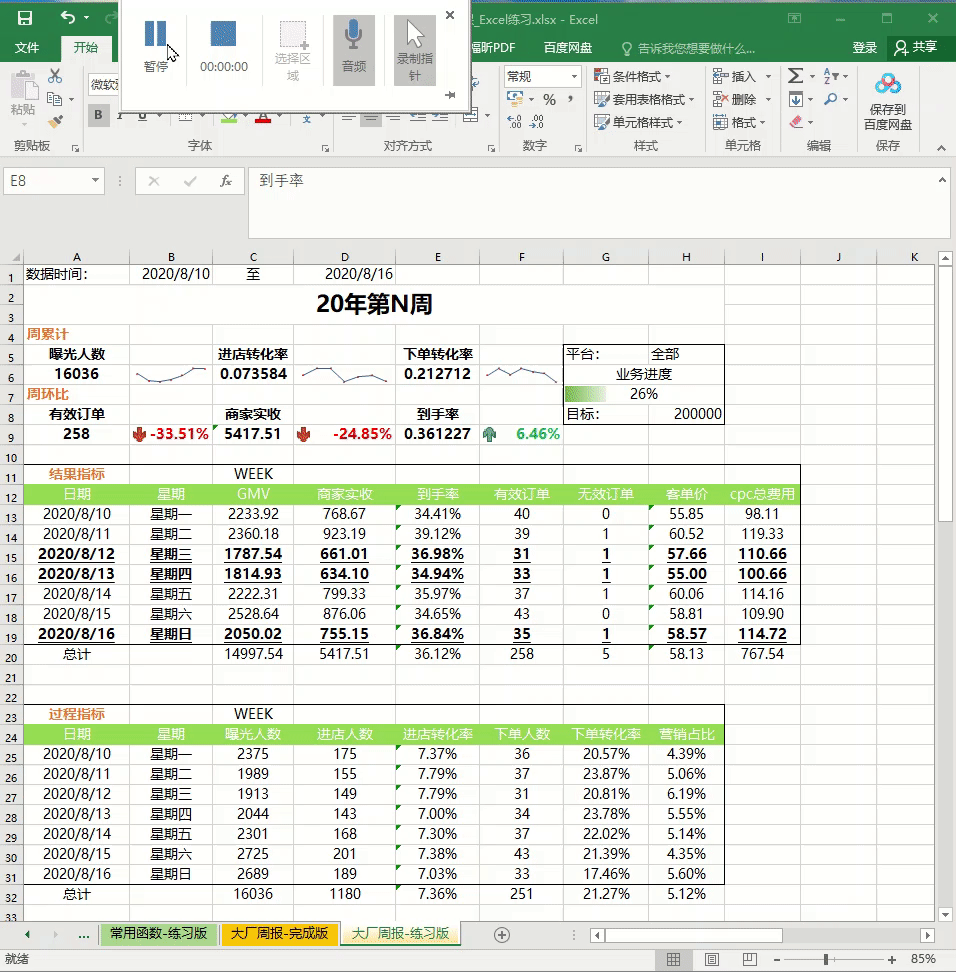# 知识点思维导图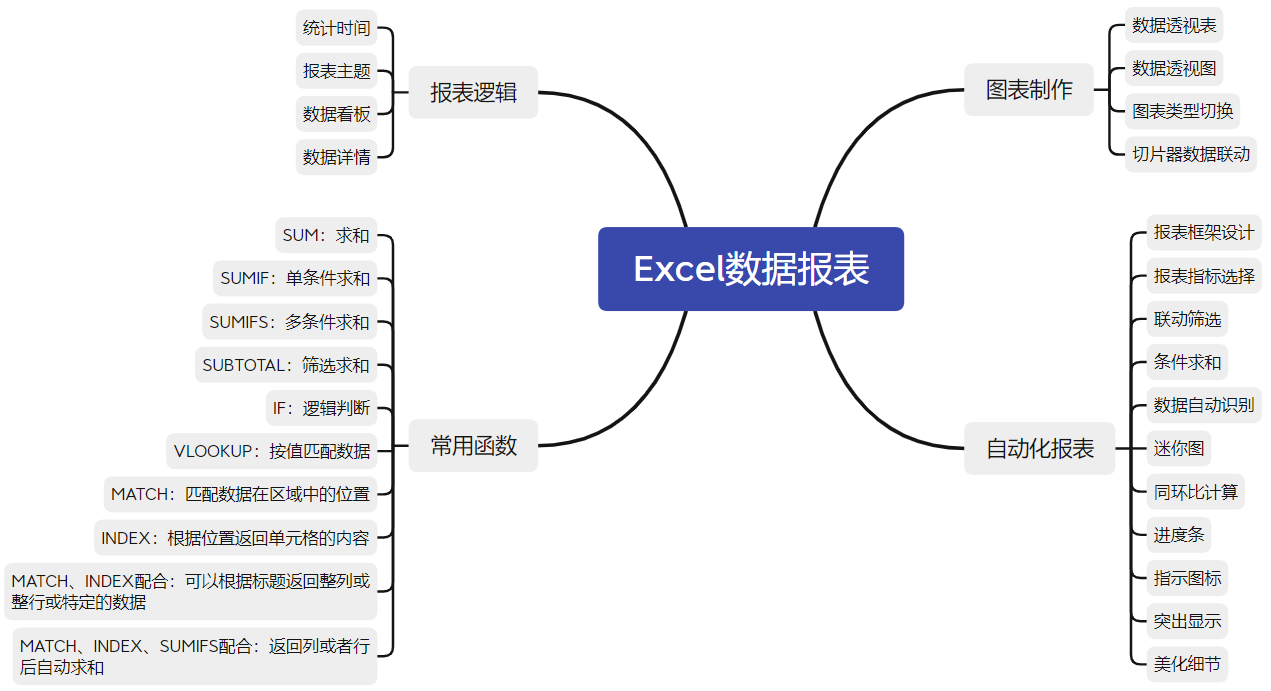# 数据透视表

## 表

1. 定义：一种交互式的数据表，可以进行特定的计算。个人认为数据透视表和普通图表的差别在于数据透视表拥有交互功能，在选择数据之后能够通过拖拽选择报表的字段，更加灵活
2. 步骤：目标定位在数据sheet–选择菜单栏插入–数据透视表
在数据透视表中即可使用拖拽选择行,列,求值等操作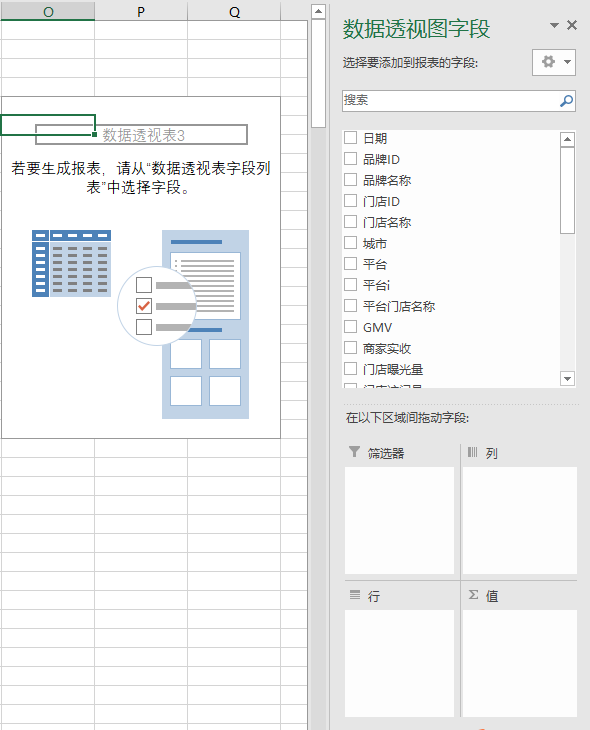## 图

1. 定义：通过选择数据透视表中的数据画图，得到的图形即为数据透视图，能根据数据透视表联动交互。
2. 步骤：选择数据透视表中的数据–插入-选择对应的图形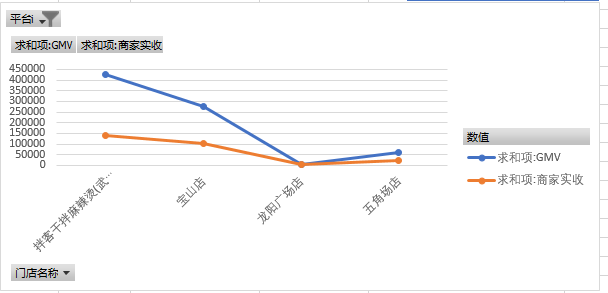## 切片器

1. 定义：切片器其实是一种筛选结构，只不过只能在数据透视表中使用，可以通过切片器选择应用在数据透视表中的数据
2. 步骤：选定数据透视表–插入–切片器–选择筛选原则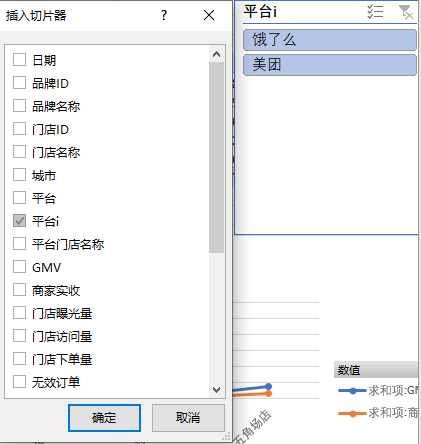# 常用函数

我把本博客中用到的函数按照功能分为求和、逻辑、匹配三个大类

## 求和函数

1. SUM：SUM(number1, number2…)，简单的求和函数，选定数值后即可通过SUM求和
2. SUMIF：SUMIF(range, criteria, [sum_range])，range是条件范围，criteria是筛选的标准，这两项是必须项，sum_range是求和的范围，可省略，省略后则对range求和。例如SUMIF(收入列, “>=&10000”, 人数列)表示选择满足收入大于10000的人数进行求和，如果省略人数列，则为SUMIF(收入列,">=&10000")表示对收入大于10000的收入进行求和
3. SUMIFS：SUMIFS(sum_range, criteria_range1, criteria1, [criteria_range2, criteria2], …) 与SUMIF基本相同，但是sum_range提到最前，新增的criteria_range1表示第一个筛选标准的范围，该函数可进行多条件选择
4. SUBTOTAL ：SUBTOTAL(function_num,ref1,[ref2],…)其中function_num参数为1-11或101-111这些数字，不同的数字代表不同的求和函数，具体如下图，ref1表示求和的数据范围；这与SUM的区别在于SUBTOTAL在筛选模式(Ctrl+Shift+L)下能够对筛选后的数据进行操作，而SUM则不受筛选器的影响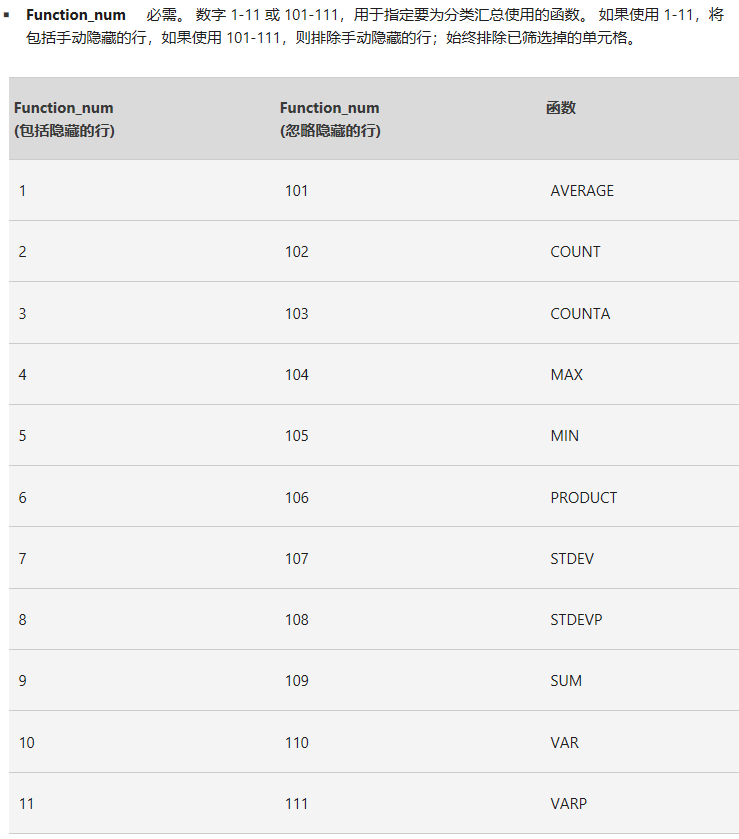## 逻辑函数

1. IF：IF(logical_test, value_if_true, [value_if_false]) 其中logical_test表示逻辑判断，后两个参数为逻辑判断为相应值时应该执行的操作

## 匹配函数

1. VLOOKUP：VLOOKUP (lookup_value, table_array, col_index_num, [range_lookup]) 其中lookup_value表示要查找的值， table_array表示查找的范围，col_index_num是一个数字，从1开始，表示需要返回的值在table_array的第几列，range_lookup表示一个逻辑值，一般为0，表示精确匹配
2. MATCH：MATCH(lookup_value, lookup_array, [match_type])其中lookup_value表示需要匹配的值，lookup_array表示寻找的范围，match_type是一个逻辑值，一般为0表示完全相等时匹配，匹配后该函数返回的是位置信息
3. INDEX：INDEX(array, row_num, [column_num]) 按照行和列返回指定位置的元素，当行为0时返回整列，当列为零时返回整行

# 自动化报表

由于Excel的公式编辑并没有注释以及分行，可读性极差，因此下文在描述具体公式时，我会用Python的规范加上注释以及换行符，以便理解整个公式的逻辑

## 报表框架

报表框架的选择需要根据具体的业务需求确定，这里所采用的数据为外卖店在美团与饿了么两家平台的数据，报表的使用者为外卖店管理人员，因此将报表的主体设定为时间与盈利性相关指标，并用迷你图与图标显示增减趋势，细节指标为具体用于分析的指标，分为结果指标与过程指标。

## 指标梳理

结果指标：以产出为导向，代表业务的最终结果

1. GMV：Gross Merchandise Volume，商品交易总额
2. 到手率：商家实收/GMV
3. CPC：Cost Per Click，每次点击付费广告，即按照点击次数付费的广告

过程指标：以投入为导向，代表业务的实际过程

1. 进店转化率：进店人数/曝光人数
2. 下单转化率：下单人数/进店人数
3. 营销占比：cpc总费用/GMV

## 联动筛选

联动筛选需要通过数据验证IF函数配合实现，如选择不同的平台是，相对应的目标也会发生改变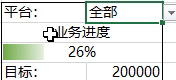1. 下拉筛选框：筛选框由数据验证实现，菜单栏–数据验证–序列–输入内容，不同的内容用英文逗号分开，如下图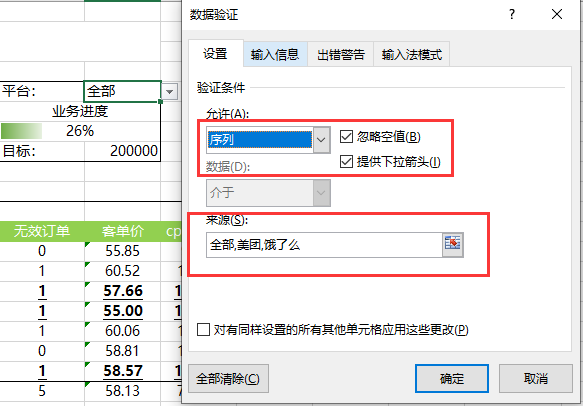2. IF函数：在目标框可以使用IF函数实现联动，如需要根据平台设置不同的目标则写法如下：
=IF(H5="全部",200000,
IF(H5="美团",150000,50000))
# Excel公式无法分行，这里为了增加可读性，用Python的格式做了分行处理，下同


## 条件求和

在条件求和这部分主要实现根据报表的日期以及行首的标题实现自动识别日期和标题然后自动求和，效果如下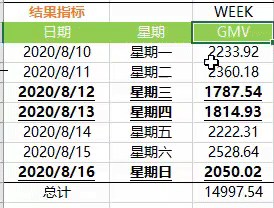写法如下：

=IF($H$5="全部", # 如果平台=全部，则执行第一个SUMIFS
SUMIFS( # 条件求和，各个参数的含义见上文求和函数部分
# INDEX函数，行号为0，即返回一整列，我们需要对这个部分的标题即GMV求和，
# 因此这个个INDEX函数的目的就是要返回源数据中的GMV列
INDEX('拌客源数据1-8月'!$A:$X,0, # 要查找的范围是整个源数据，全选，行号选择0
MATCH(C$12,'拌客源数据1-8月'!$1:$1,0)), # 列号要根据报表的标题选择，C$12为报表中“GMV”字段的位置，第二个参数为源数据的第一行，第三个参数为0表示精确匹配，则MATCH函数就会在源数据的第一行找到“GMV”字段的列号
# 这是的求和条件，报表需要的是当天的GMV总和，因此此处的INDEX函数需要返回的是日期列，将其作为求和的条件范围
INDEX('拌客源数据1-8月'!$A:$X,0,
MATCH($A$12,'拌客源数据1-8月'!$1:$1,0)),
$A13), # 当平台不等于全部时，执行以下步骤 SUMIFS( # 同样返回源数据中的GMV列 INDEX('拌客源数据1-8月'!$A:$X,0, MATCH(C$12,'拌客源数据1-8月'!$1:$1,0)),
# 同上，返回日期列，这是第一个筛选条件
INDEX('拌客源数据1-8月'!$A:$X,0,
MATCH($A$12,'拌客源数据1-8月'!$1:$1,0)),
$A13, # 第二个筛选条件，返回平台列，根据平台筛选，因为这里平台不是全部了 INDEX('拌客源数据1-8月'!$A:$X,0, MATCH("平台i",'拌客源数据1-8月'!$1:$1,0)),$H$5))  ## 数据自动识别 上文的条件识别其实就已经实现了数据自动识别，但是需要注意的点在于我们需要对条件识别中某些单元格的选取做处理，因为在Excel拖拽填充时，选择的单元格会发生偏移。例如要返回日期列时，我们采用的公式如下 INDEX('拌客源数据1-8月'!$A:$X,0, MATCH($A$12,'拌客源数据1-8月'!$1:$1,0)),$A13,


公式中的美元符号$表示绝对引用，即我们做下拉填充时，这个值不会发生改变，如果没有美元符号，当我们下拉时，A13会变为A14，右填充时A13会变成B13，因此为了不让A发生改变，需要加上美元符号，公式中出现的美元符号都是同样的作用。 因此个人认为，数据自动识别的关键点除了INDEX和MATCH两个函数的配合外，还需要$的配合

## 迷你图

迷你图和普通的图形没什么本质上的差别，只是迷你图只占用一个单元格而已，方法和普通的图形一样：选择数据–插入–迷你图–选择图形即可

## 进度条、图标指示、突出显示

这三者都属于条件格式的应用

• 进度条其实是单元的规则，使用方法为：开始–条件格式–基于各自值…–格式样式选择数据条–最小值和最大值设置–外观设置。除了进度条还可以设置双色三色刻度等，在本文的报表中，需要体现进度，所以采用数据条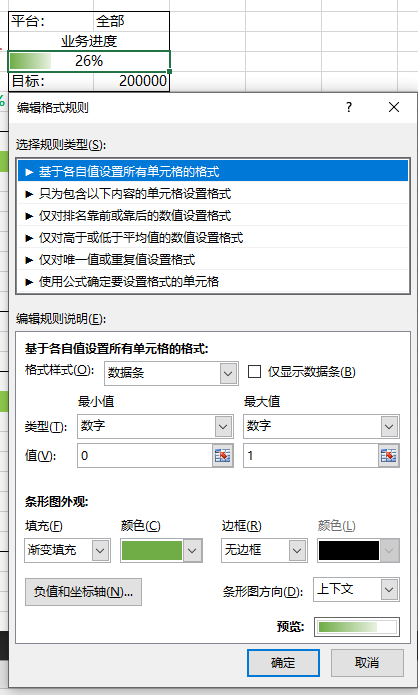• 图标指示也是条件格式中的一种，与进度条相同，只是在格式样式选择时，选择图标集，再对不同的数值选择不同的图标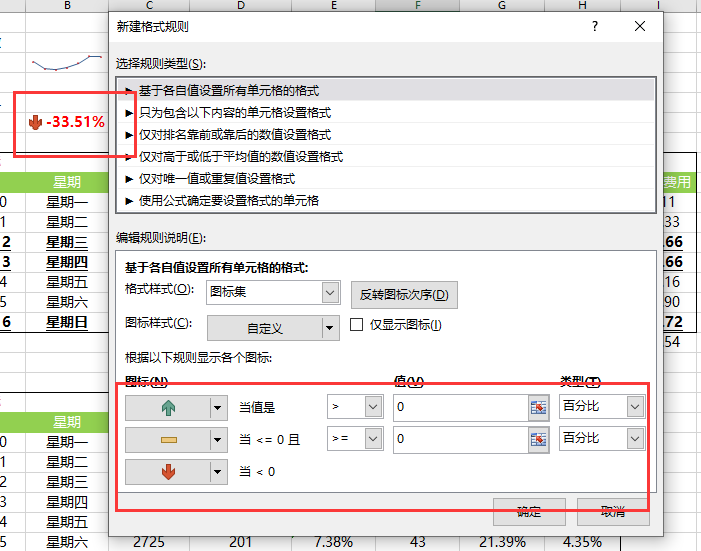• 突出显示同样属于条件格式的一种，在本文中的体现即当天GMV低于本周平均值时，该行的内容会加粗并添加下划线，操作步骤与进度条相同，只是规则类型为使用公式确定要设置格式的单元格，接下来在规则说明里写上所需公式即可，这里的公式支持Excel所有自带函数，在格式中可以设置满足规则的字体样式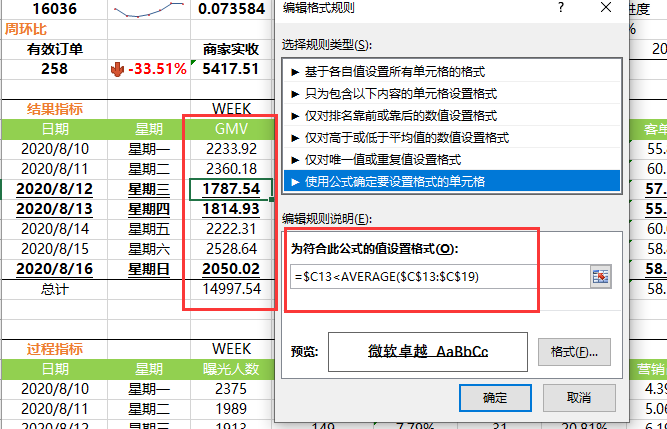# 总结

• 首先感谢B站戴师兄分享的报表制作视频，INDEX，MATCH两个函数配合确实可以产生很美妙的效果，思路很棒
• 个人认为周报的制作应该尽量实现自动化，作为数据分析人员应该把更多的精力放在数据的建模、分析、归因等数学以及业务上，而不是单纯的做个SQL、报表Boy，掌握Excel这个工具的高级用法也是为了更好地为数据分析服务。
• 编写Excel公式时，由于没有换行和注释功能，直接在Excel上写会非常心累，括号的匹配简直看花眼，可以在稿纸上先写好伪代码，然后再把相应的单元格填上，先思考，后动手。
展开全文• Excel动态数据报表制作

# 一、基本概念

1.表格组成：工作簿-工作表-行/列-单元格
2.功能区
（空闲时可逐一进行了解）鼠标悬停在功能区某一按钮上即会出现该功能注释# 二、基础操作

• 源数据备份
拿到一份数据之后，第一步操作进行备份，以免对数据造成不可还原的修改。
操作：选中源数据工作表-【右键】选择【移动或复制工作表】-选中【移至最后】并勾选【创建副本】-选中副本-【右键】【重命名】为“源数据备份”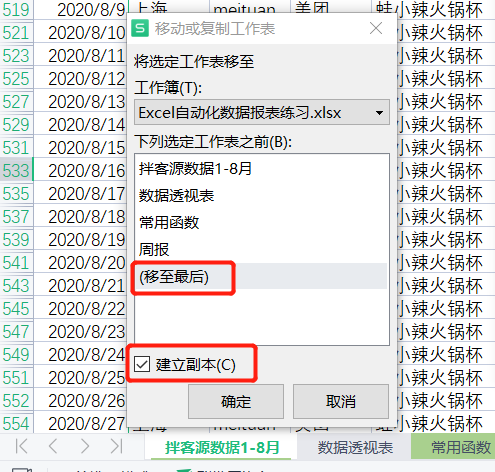# 三、理解数据

## 1.数据量级

• 选中整行：整个数据一共24列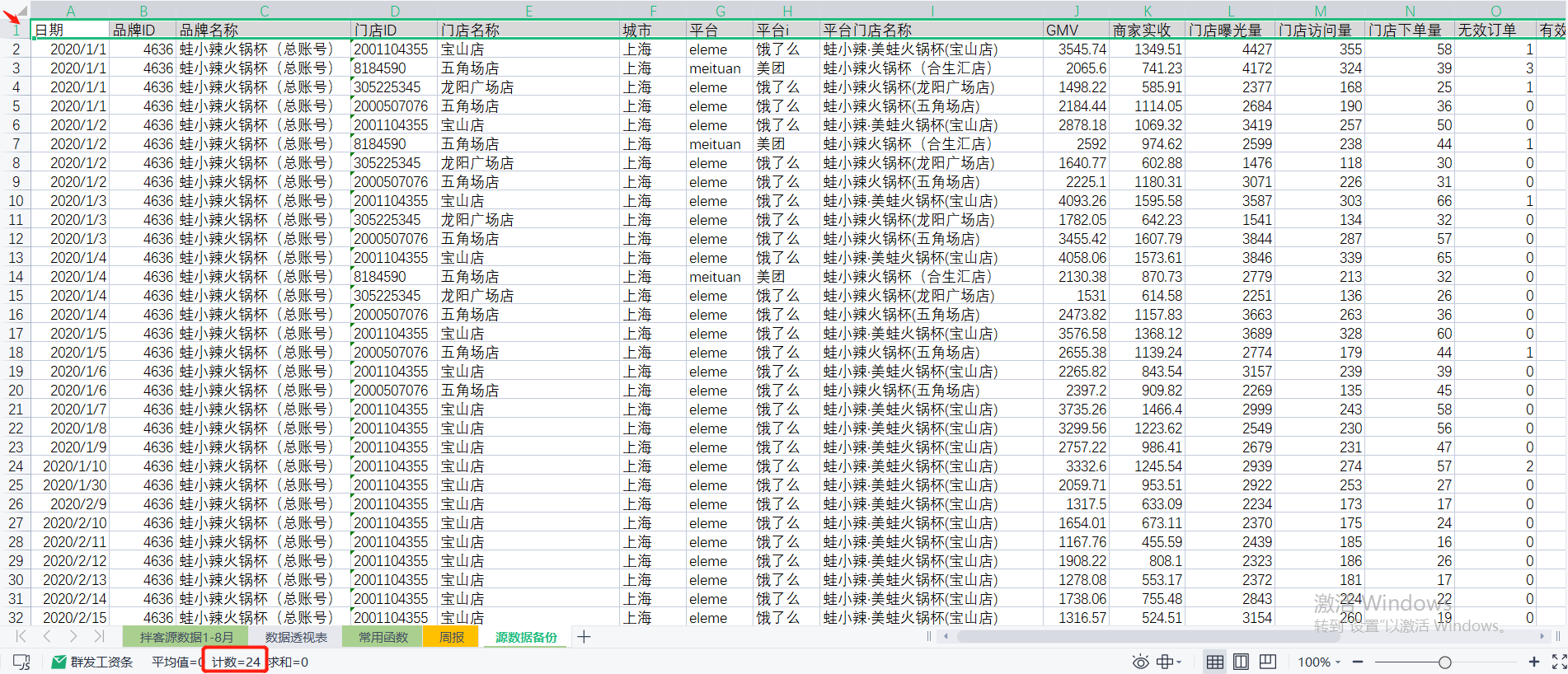• 选中整列：整个数据一共562行，除去表头数据实际一共521行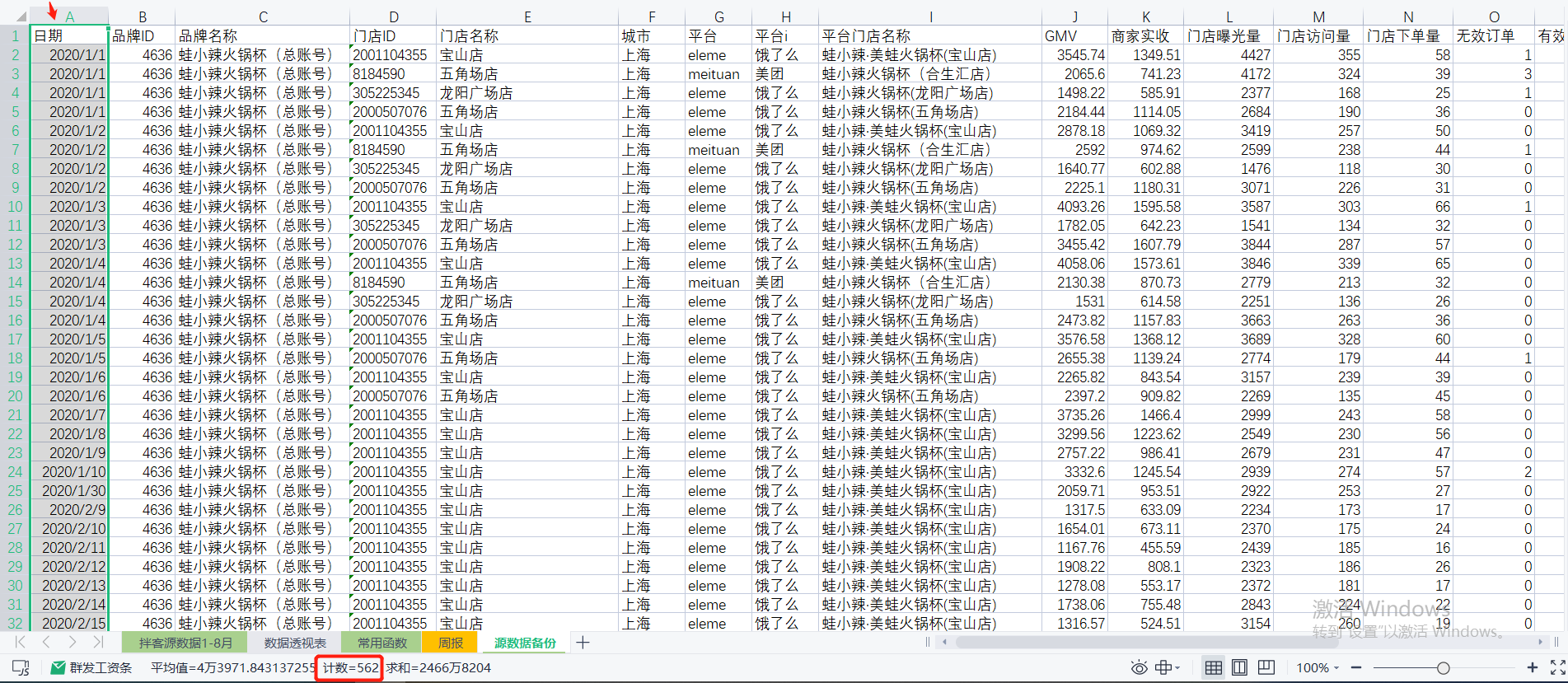## 2.数据类型

注:该报表使用的数据为线上外卖门店的数据

• 日期:指的是一家外卖门店当天所有营业额所归属的日期；
• 品牌ID:相当于品牌身份证号
• 品牌名称:门店所在品牌名称
Ctrl+Shift+L快速筛选，可以快速了解门店品牌数、排序、筛选等；
• 门店ID:相当于门店的身份证号
• 门店名称
• 城市
• 平台:平台的拼音格式
• 平台i:平台的中文格式
• 平台门店名称
注：同样一家店，存在不一样的格式（如:拌客干拌麻辣烫(武宁路店)→拌客干拌麻辣烫（武宁路店）→拌客·干拌麻辣烫(武宁路店)，以增加空格、点的形式区分店名），原因是该门店存在关店重开的情况，每次关店重开后会更换平台门店名称以此与之前的门店做区分，更方便的收集数据。
• GMV:全称为GrossMerchandiseVolume即商品交易总额，是一段时间内的成交总额的意思，多用于电商行业，一般包含拍下未支付订单金额（未刨除各项补贴的表面金额）。
• 商家实收:去除平台补贴、商家补贴、红包、满减、配送费、平台抽成等等后，当天实际可以进入银行卡/平台账户收入里的金额
• 门店曝光量:指的是该门店在外卖平台被用户看到的次数
• 门店访问量:用户进入该门店的次数
• 门店下单量
• 无效当订单
• 有效订单
• 曝光人数：对应去重的曝光量
• 进店人数：对应去重的访问量
• 下单人数：对应去重的下单量
• cpc总费用:CPC(Cost Per Click)每产生一次点击所花费的成本。在这个报表中cpc总费用指的是当天广告投放的总花费
• cpc曝光量:广告投放为门店带来的曝光量
• cpc访问量:广告投放为门店带来的访问量
• 商户补贴:商家补贴金额
• 平台补贴：平台补贴金额

## 3.数据含义

### 环比

环比=(本期数/上期数)/上期数=本期数/上期数-1


1)年环比

2020年环比=(2020年数据-2019年数据)/2020年数据=2020年数据/2019年数据-1


2)月环比

2020年7月环比=2020年7月数据/2020年6月数据-1


3)日环比

2020年7月1日环比=2020年7月1日数据/2020年6月30日数据-1


### 同比

同比=(本期数-同期数)/本期数=本期数/同期数-1


1)月同比

2020年7月同比=2020年7月数据/2019年7月数据-1


2)日同比

2020年7月1日的月同比=2020年7月1日数据/2020年6月1日数据-1
2020年7月1日的周同比=2020年7月1日数据/2020年6月24日数据-1


# 四、常用函数

1.sum：可以对整行、整列、一个区域进行求和

2.sumif：单条件求和

sumif(range,criteria,[sum_range])
sumif(条件判断所在的区域,条件,[用来求和的数值区域])


3.sumifs：多条件求和

sumifs(sum_range,[criteria_range1],[criteria1],[criteria_range2],[criteria2],..)
sumifs(用来求和的数值区域,条件1判断所在的区域1,条件1,条件2判断所在的区域1,条件2,...)


例：求整月美团平台的GMV

=sumifs(GMV列,平台列,"美团",日期列,">="&每月第一天,日期列,"<="&每月最后一天)


注：条件参数直接引用单元格或者使用函数不需要英文双引号，若使用如"美团"这样的字符串做条件参数，需要加英文双引号；以及大于等于号也需要添加英文双引号，并且使用&才能与后面的条件值相连。

4.subtotal：根据筛选求和、平均等等（11个函数,以数字区分，9为sum函数）

subtotal(function_num,ref1,[ref2],...)
subtotal(指定函数，选择区域1，[选择区域2],...)


例如：

subtotal(9,GMV列)=sum(GMV列) 仅当全选时该等式成立。


5.Year:提取日期的年，以数字格式显示

YEAR(serial_number) YEAR(日期)


6.Month:提取日期的月

MONTH(serial_number) MONTH(日期)


7.DAY:提取日期的天

DAY(serial_number) DAY(日期)


8.DATE:组合年月日

DATE(year,month,day) DATE(代表年份的数值，代表月份的数值，代表日份的数值)


与YEAR()、MONTH()、DAY()组合，可用来求得上一年、上月、上周、昨天、下一年、下个月、下周、明天等对应日期。例如：

每个月的第一天=DATE(year(日期),month(日期),1)；
每个月的最后一天=下个月的第一天-1=DATE(year(日期),month(日期)+1,1) -1


注：不要使用excel里的日期格式存储日期，建议使用字符串形式存储日期，否则将表格导入数据库会出现其他问题

8.if：逻辑判断

if(logical_test,value_if_true,[value_if_false])
if(逻辑比较条件,结果成立时返回的值,[结果不成立时返回的值])
[value_if_false]:该参数选填，没有该参数时，返回False


例：利用if函数嵌套，判断A、B是否为0

if(A=0,if(B=0,"AB都为0","A等于0，B不等于0"),if(B=0,"A不等于0，B等于0","AB都不等于0"))


9.vlookup：连接匹配数据

VLOOKUP(lookup_value,table_array,col_index,[range_lookup])
VLOOKUP(要查找的数据,要查找的位置和要返回的数据的区域,要返回的数据在区域中的列号,返回近似匹配或精确匹配-1/true或0/false)

• 要查找的位置必须在区域的第一列，否则会报错
• VLOOKUP只会返回它查找到的第一个值
• VLOOKUP的模糊匹配非通常的模糊匹配，使用VLOOPUP模糊匹配文本类型数据时可以配合通配符使用。
通配符：
*:代替不定数量的字符
？:(英文输入状态下)代替一个字符


配合通配符进行模糊匹配（第三个参数为0）：

返回以A开头的数据对应的第二列的值=VLOOKUP("A*",区域,2,0)
返回以A开头的三个字符对应的第二列的值=VLOOKUP("A??",区域,2,0)


10.match：查找数值在区域中的位置

MATCH(lookup_value,lookup_array,[match_type])
MATCH(查找项,查找区域,0)


例：返回A在第几行

=MATCH("A",A所在列,0)


返回A在第几列

=MATCH("A",A所在行,0)


11.index：根据区域的位置返回数值

INDEX(array,row_num,column_num)
INDEX(区域，行号，列号)


12.match、index一起使用：自动根据列名查找数据
制作报表时，可以利用match函数在源数据中找到报表表头对应的数据列号或行号，结合Index函数找到对应的列/行进行后续计算。
例：图一为源数据表，图二为数据报表，根据表头信息在源数据中找到对应数据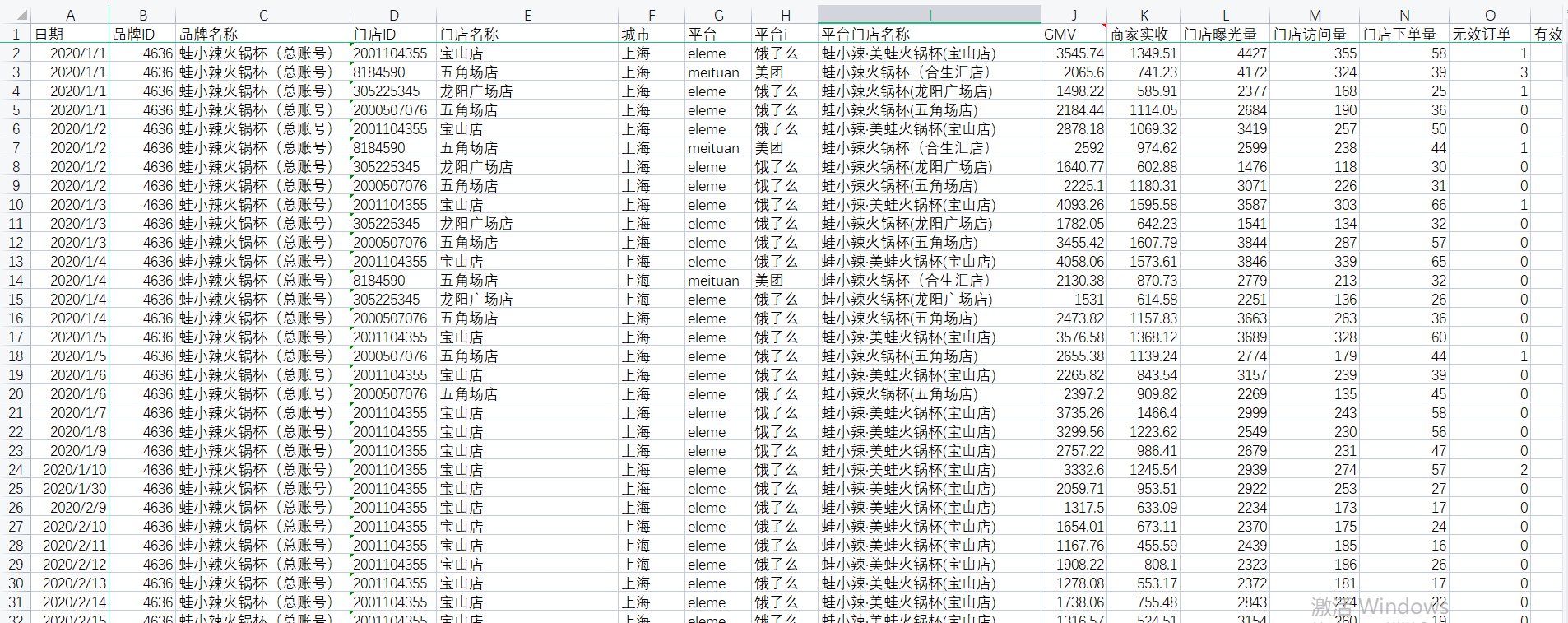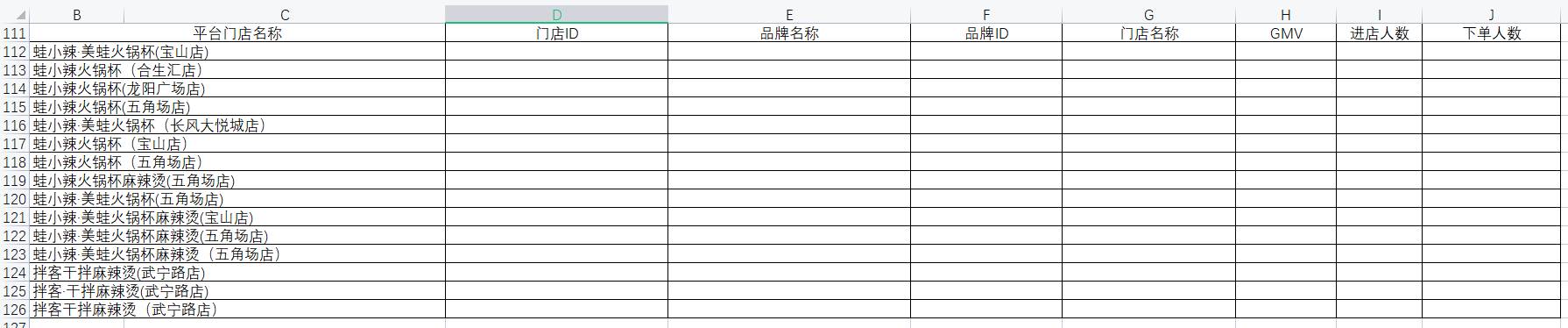过表头查找【蛙小辣·美蛙火锅杯(宝山店)】即【B112】单元格对应的门店ID：

• 因为要查找的数据在源数据表中，所以INDEX函数第一个区域参数为源数据所有列，即
'源数据表'!A:X    （!表示非本工作表）

• 【蛙小辣·美蛙火锅杯(宝山店)】的门店ID与该门店的平台店名称在同一行，所以查找门店ID的行号等同于查找【蛙小辣·美蛙火锅杯(宝山店)】在源数据表的行号。【蛙小辣·美蛙火锅杯(宝山店)】在【平台店名称】列，即I列，使用MATCH定位行号，即
MATCH(B112,'源数据表'!I:I,0)

• 【蛙小辣·美蛙火锅杯(宝山店)】门店ID的列，即查找【门店ID】即【D111】单元格在源数据的列号，【门店ID】在第一行，使用MATCH定位列号，即
MATCH(D111,'源数据表'!1:1,0)

• 完整函数格式为：
=INDEX('源数据表'!A:X,MATCH(B112,'源数据表'!I:I,0),MATCH(D111,'源数据表'!1:1,0))


编写好单个函数后，希望通过下拉、右拉可以自动查找对应的其他值。但是，下拉后会出现部分匹配不到数据的情况。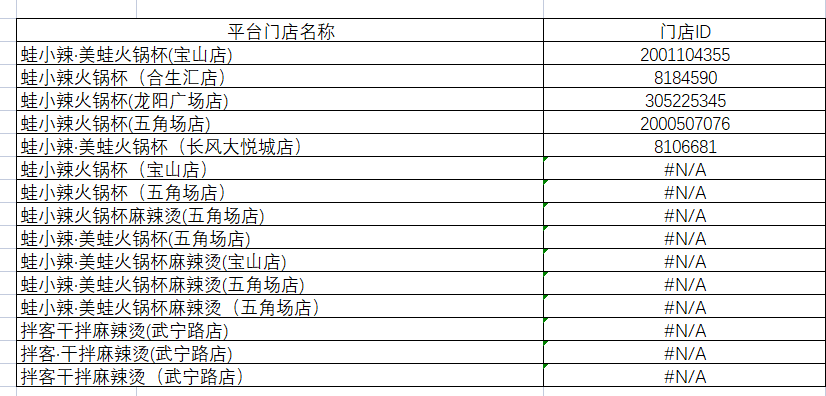因为下拉的同时，单元格的选中区域【蛙小辣·美蛙火锅杯(宝山店)】、【门店ID】等会依次向下移动，因此需要固定部分选中区域，让我们在下拉、右拉的同时保持不变。使用美元符号"$"进行锁定操作。 1）源数据表各区域固定，均需锁住； 2）[B112]需根据下拉向下拉匹配其他平台门店名称数据，同时右拉时固定该列，因此只需锁定B列,即$B112；
3）[D111]需根据右拉匹配其他表头数据，同时下拉时固定该行，即 D$111  • 完整函数格式为： =INDEX('源数据表'!$A:$X,MATCH($B112,'源数据表'!$I:$I,0),MATCH(D$111,'源数据表'!$1:$1,0))  13.sumifs、match、index顶级用法：自动根据列名的数据和条件进行求和 INDEX函数中，当行号为0时，返回一整列，当列号为0时返回一整行，因此通过MATCH返回查找值在源数据表中时第几行/列，再通过INDEX定位整列区域，结合sumifs函数进行条件筛选求和计算。 GMV、进店人数、下单人数均用sumifs、match、index结合的方式计算。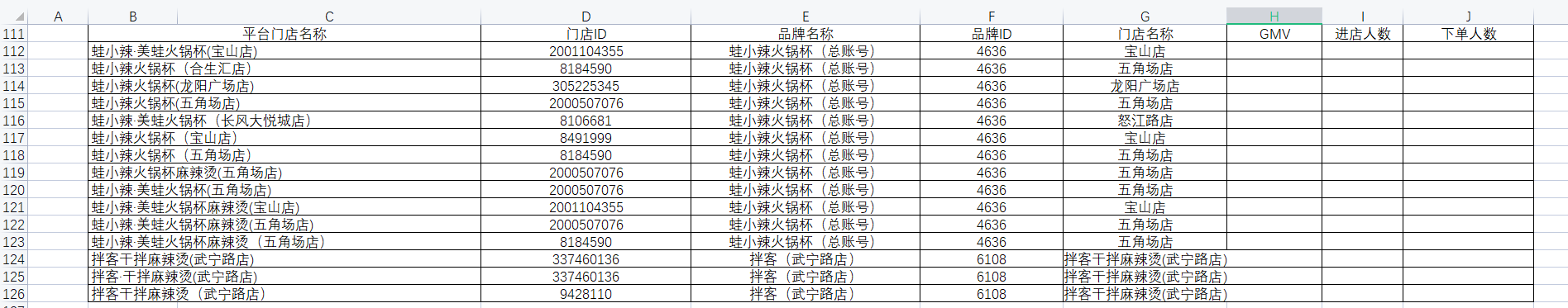求GMV • 利用INDEX、MATCH函数取得求和区域GMV列、取得条件判断区域平台店名称列： GMV列=INDEX('源数据表'!$A:$X,0,MATCH(H$111,'源数据表'!$1:$1,0))
平台店名称列=INDEX('源数据表'!$A:$X,0,MATCH($B$111,'源数据表'!$1:$1,0))

• 组合sumifs函数求出平台门店对应GMV总和
=SUMIFS(INDEX('源数据表'!$A:$X,0,MATCH(H$111,'源数据表'!$1:$1,0)),INDEX('源数据表'!$A:$X,0,MATCH($B$111,'源数据表'!$1:$1,0)),$B112)

• 通过向下拉动、向右拉动可自动得出门店下单人数总和、下单人数总和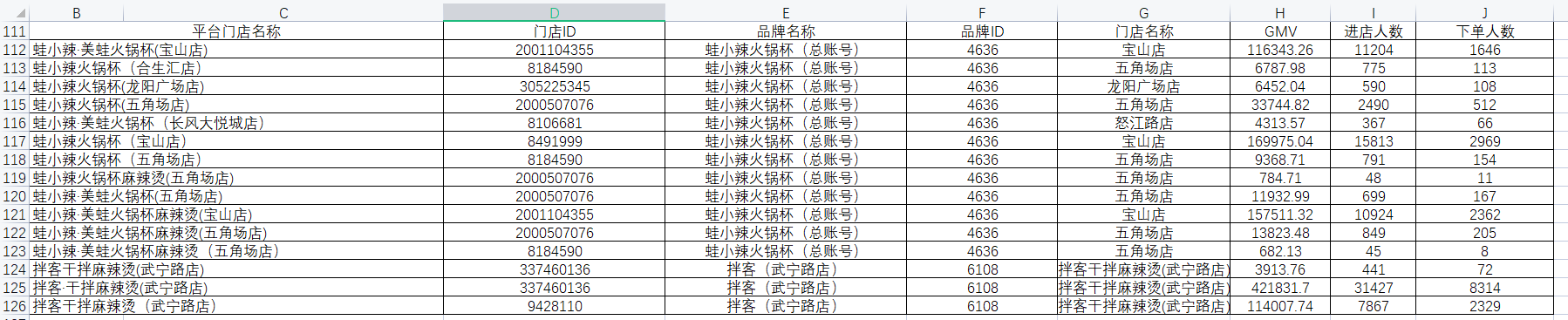# 五、自动化报表开发

## 1.报表框架

### 1)整体分布

一共分为四个部分：标题、小看板（含目标）、结果指标、过程指标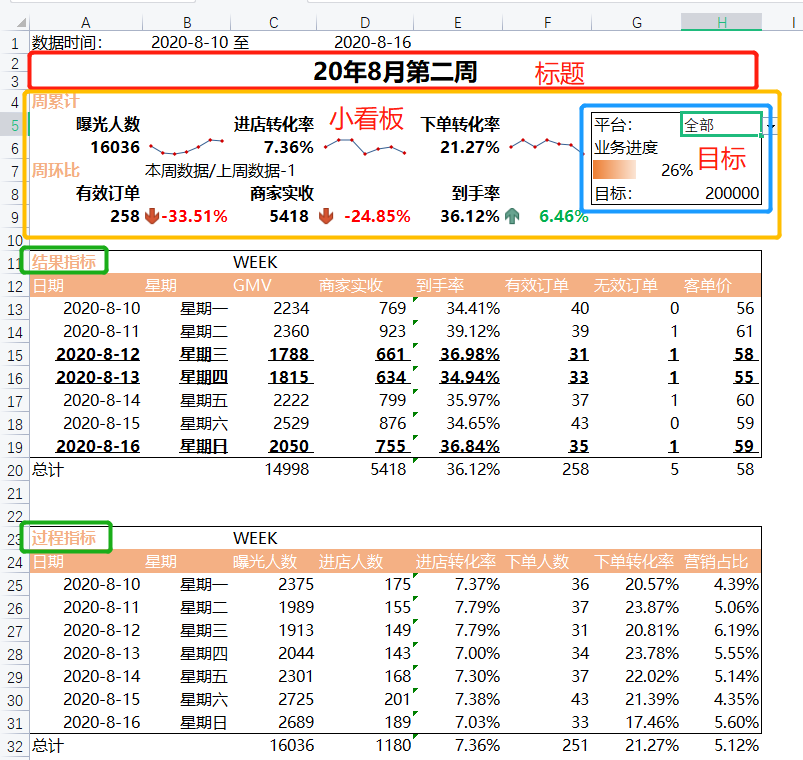### 2)时间信息

①日期
日期只需填写一个，其他日期均再次日期的基础上引用，可以极大提升效率，更改一个日期可以联动更新整个工作表的数据。
过程指标中的日期也是通过引用该日期实现。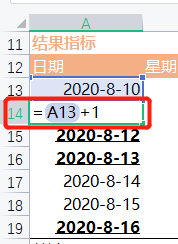②星期
同样引用上一个日期，更改格式即可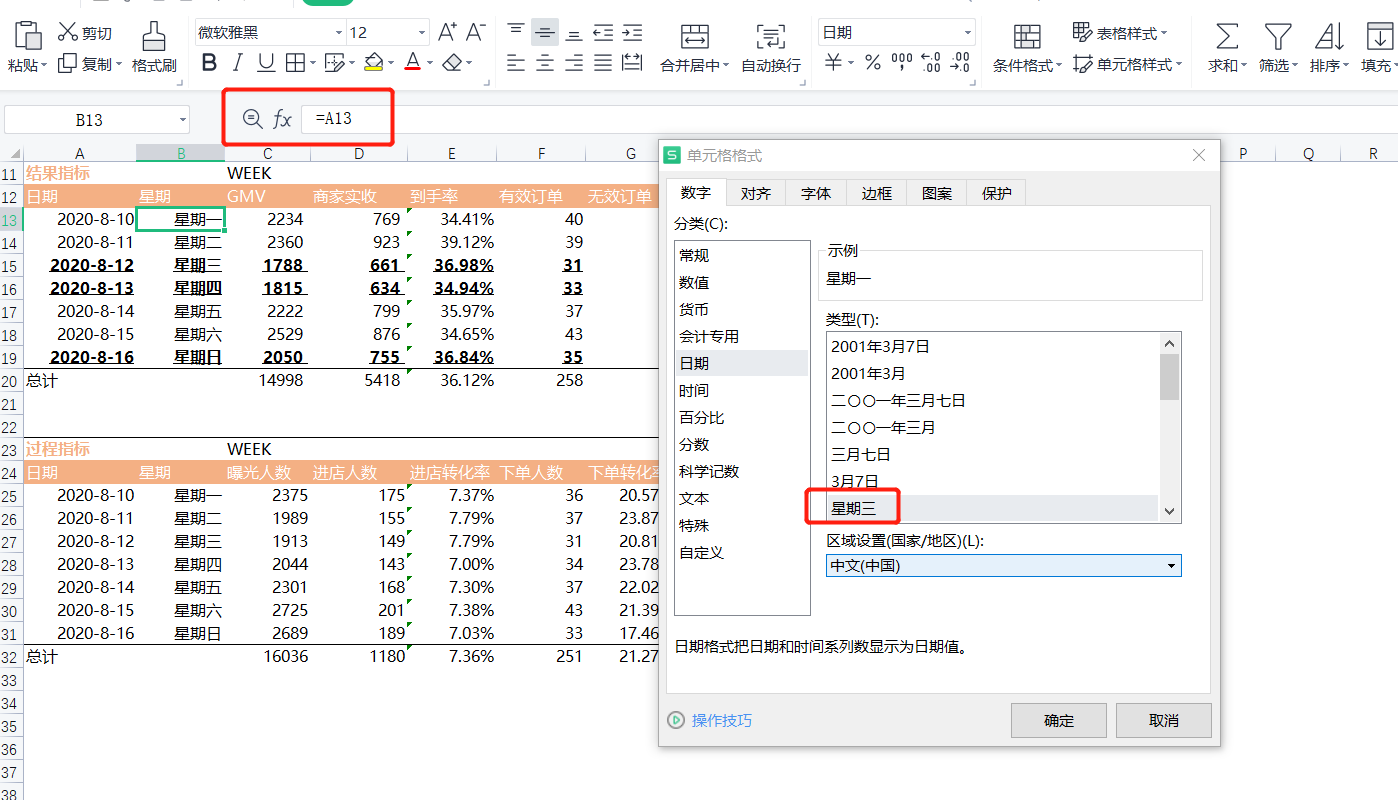③数据时间
同样进行日期的引用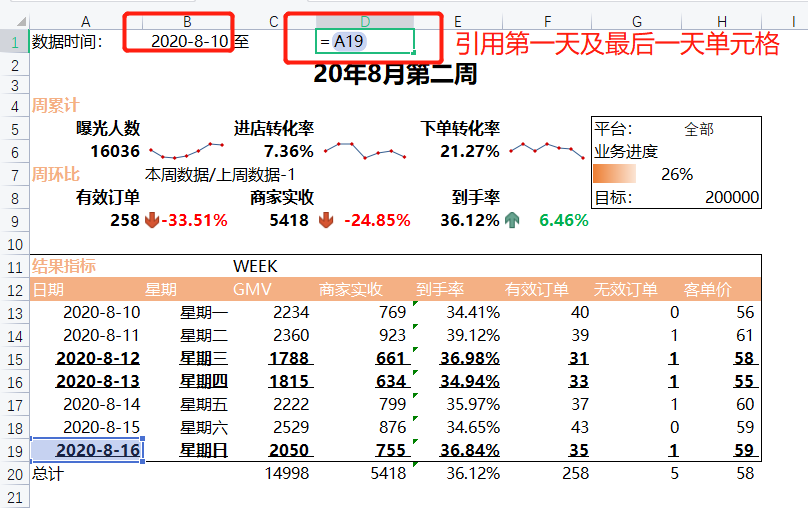## 2.指标梳理

### 1)结果指标

与商家收入的相关指数
GMV、商家实收、到手率(商家实收/GMV)、有效订单、无效订单、客单价(GMV/有效订单)

### 2)过程指标

商家经营情况的直观体现
曝光人数、进店人数、进店转化率(进店人数/曝光人数)、下单人数、下单转化率(下单人数/进店人数)、营销占比(cpc总费用/GMV)

## 3.联动筛选

1)下拉筛选器
在小看板中目标位置，设置了一个可供切换平台的筛选器，实际上它是一个条件验证，其他函数通过判断当前所选平台来计算对应数据。因此需要先设置该筛选器，后续判断一律引用该筛选器单元格，实现切换平台联动更新数据表。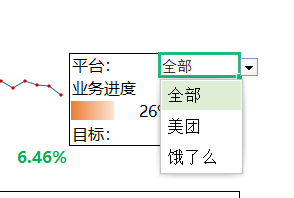对应操作：【数据】→【数据验证or数据有效性】→选择【序列】→输入【全部,美团,饿了么】注意使用英文逗号→【确定】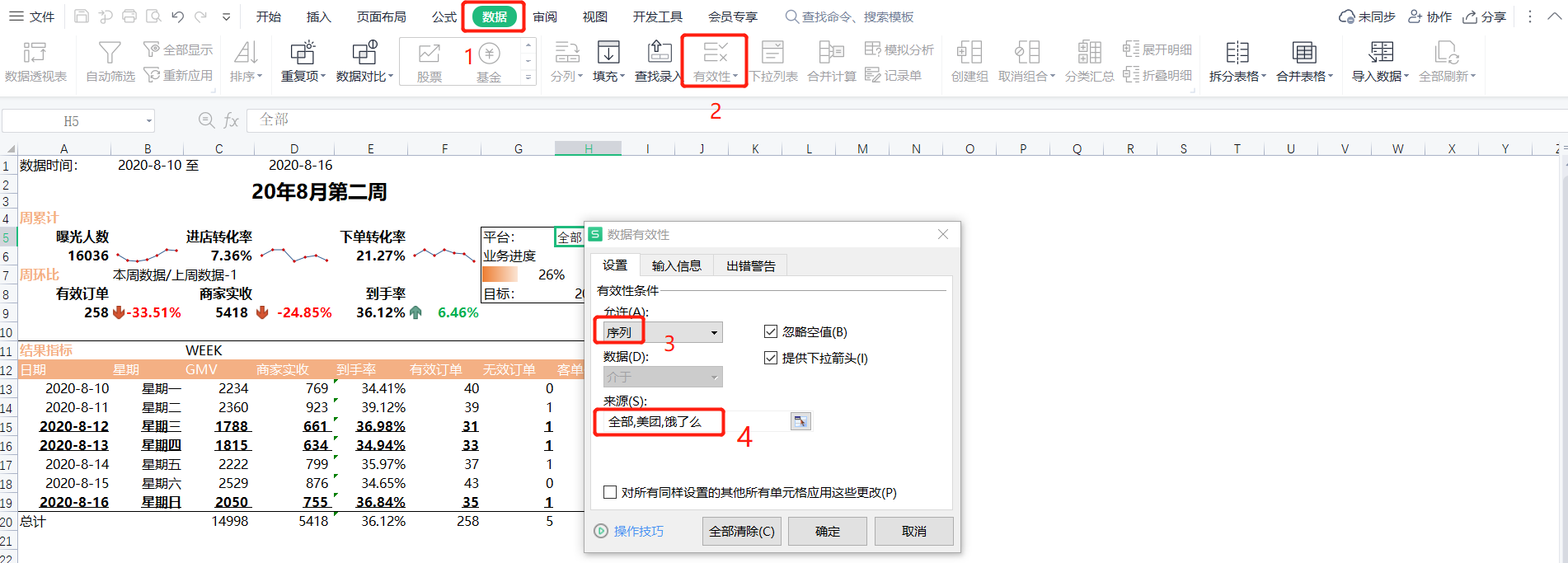## 4.条件求和

### 1)if、sumif、sumifs函数嵌套逻辑

求和GMV指标，需判断当前平台以及日期筛选。
if函数判断当前平台是全部、美团还是饿了么，当平台为全部时，无需进行平台筛选，使用sumif函数按日期筛选GMV求和即可；当平台为美团或饿了么时，需使用sumifs函数同时进行平台筛选及日期筛选GMV求和。

### 2)条件求和函数实现

if(平台=全部,sumif(日期列,日期,GMV列),sumifs(GMV列,日期列,日期,平台列,平台))


为了实现最大的灵活程度，使用index根据表头查找对应源数据列，只编写一此，通过向下向右拖拽即可自动填充其他求和项。

• 日期列
INDEX('源数据'!$A:$X,0,MATCH($A$12,'源数据表'!$1:$1,0))

• GMV列（求和列）
INDEX('源数据表'!$A:$X,0,MATCH(C$12,'源数据表'!$1:$1,0))  • 平台列 INDEX('源数据表'!$A:$X,0,MATCH("平台i",'源数据表'!$1:$1,0))  • 完整函数实现 =IF($H$5="全部",SUMIF(INDEX('源数据'!$A:$X,0,MATCH($A$12,'源数据表'!$1:$1,0)),$A13,INDEX('源数据表'!$A:$X,0,MATCH(C$12,'源数据表'!$1:$1,0))),SUMIFS(INDEX('源数据表'!$A:$X,0,MATCH(C$12,'源数据表'!$1:$1,0)),INDEX('源数据表'!$A:$X,0,MATCH($A$12,'源数据表'!$1:$1,0)),$A13,INDEX('源数据表'!$A:$X,0,MATCH("平台i",'源数据表'!$1:$1,0)),$H$5))  将该函数应用到过程指标中的【曝光人数】，只需将求和列中的GMV单元格修改为曝光人数对应的单元格即可，其他不变。 INDEX('源数据表'!$A:$X,0,MATCH(C$24,'源数据表'!$1:$1,0))

• 【曝光人数】完整函数实现
=IF($H$5="全部",SUMIF(INDEX('源数据表'!$A:$X,0,MATCH($A$24,'源数据表'!$1:$1,0)),$A25,INDEX('源数据表'!$A:$X,0,MATCH(C$24,'源数据表'!$1:$1,0))),SUMIFS(INDEX('源数据表'!$A:$X,0,MATCH(C$24,'源数据表'!$1:$1,0)),INDEX('源数据表'!$A:$X,0,MATCH($A$24,'源数据表'!$1:$1,0)),$A25,INDEX('源数据表'!$A:$X,0,MATCH("平台i",'源数据表'!$1:$1,0)),$H$5))

• 商家实收、有效订单、无效订单均可通过GMV指标右拉实现数据自动填充；
• 到手率=商家实收/GMV客单价=GMV/有效订单，这两个指标进行简单除法运算即可；
• 进店人数、下单人数均可通过曝光人数指标右拉实现数据自动填充；
• 进店转化率=进店人数/曝光人数下单转化率=下单人数/进店人数，这两个指标同样进行简单除法运算即可；
• 营销占比=cpc总费用/GMV。但是当前数据报表中没有展示cpc总费用的数据，需要额外进行计算，计算方式同求GMV的函数实现完全一致，只需将GMV单元格C12修改为"cpc总费用"(注意中文字符串需加上英文格式双引号)即可，再引用结果指标中已经计算好的GMV数据即可。完整函数实现如下：
=IF($H$5="全部",SUMIF(INDEX('源数据表'!$A:$X,0,MATCH($A$12,'源数据表'!$1:$1,0)),$A13,INDEX('源数据表'!$A:$X,0,MATCH("cpc总费用",'源数据表'!$1:$1,0))),SUMIFS(INDEX('源数据表'!$A:$X,0,MATCH("cpc总费用",'源数据表'!$1:$1,0)),INDEX('源数据表'!$A:$X,0,MATCH($A$12,'源数据表'!$1:$1,0)),$A13,INDEX('源数据表'!$A:$X,0,MATCH("平台i",'源数据表'!$1:$1,0)),$H$5))/C31


### 3)总计计算

• GMV、商家实收、有效订单、无效订单指标的总计，使用sum函数计算即可；
• 总计的到手率、客单价依旧使用简单除法计算；
• 曝光人数、进店人数、下单人数指标的总计，同样使用sum函数计算即可；
• 总计的进店转化率、下单转化率依旧使用简单除法计算；
• 总计的营销占比=一周的cpc总费用总计/一周的GMV总计。GMV总计可以从结果指标中引用C20单元格，cpc总费用总计需额外进行计算。计算一周的cpc总费用，只需要再计算一天cpc总费用函数的基础上，将单个的日期筛选修改为两个日期的大小判断，大于等于当周的第一天，小于等于当周的最后一天，通过引用日期列的开始日期单元格A13、结束日期单元格A19即可。逻辑如下
if(平台=全部,sumifs(cpc总费用列,日期列,">="&开始日期,日期列,"<="&结束日期),sumifs(cpc总费用列,日期列,">="&开始日期,日期列,"<="&结束日期,平台列,平台))

• cpc总费用总计完整函数实现
IF($H$5="全部",SUMIFS(INDEX('源数据表'!$A:$X,0,MATCH("cpc总费用",'源数据表'!$1:$1,0)),INDEX('源数据表'!$A:$X,0,MATCH($A$12,'源数据表'!$1:$1,0)),">="&$A13,INDEX('源数据表'!$A:$X,0,MATCH($A$12,'源数据表'!$1:$1,0)),"<="&$A19),SUMIFS(INDEX('源数据表'!$A:$X,0,MATCH("cpc总费用",'源数据表'!$1:$1,0)),INDEX('源数据表'!$A:$X,0,MATCH($A$12,'源数据表'!$1:$1,0)),"<="&$A13,INDEX('源数据表'!$A:$X,0,MATCH($A$12,'源数据表'!$1:$1,0)),"<="&$A19,INDEX('源数据表'!$A:$X,0,MATCH("平台i",'源数据表'!$1:$1,0)),$H$5))

• 总计的营销占比完整函数实现
=IF($H$5="全部",SUMIFS(INDEX('源数据表'!$A:$X,0,MATCH("cpc总费用",'源数据表'!$1:$1,0)),INDEX('源数据表'!$A:$X,0,MATCH($A$12,'源数据表'!$1:$1,0)),">="&$A13,INDEX('源数据表'!$A:$X,0,MATCH($A$12,'源数据表'!$1:$1,0)),"<="&$A19),SUMIFS(INDEX('源数据表'!$A:$X,0,MATCH("cpc总费用",'源数据表'!$1:$1,0)),INDEX('源数据表'!$A:$X,0,MATCH($A$12,'源数据表'!$1:$1,0)),"<="&$A13,INDEX('源数据表'!$A:$X,0,MATCH($A$12,'源数据表'!$1:$1,0)),"<="&$A19,INDEX('源数据表'!$A:$X,0,MATCH("平台i",'源数据表'!$1:$1,0)),$H$5))/C20


## 5.迷你图

小看板中周累计的曝光人数、进店转化率、下单转化率直接引用过程指标数据即可。
插入迷你图操作
【选中整周数据】→菜单【插入】→功能区【折线】→选中迷你图放置区域→【确定】→勾选【标记】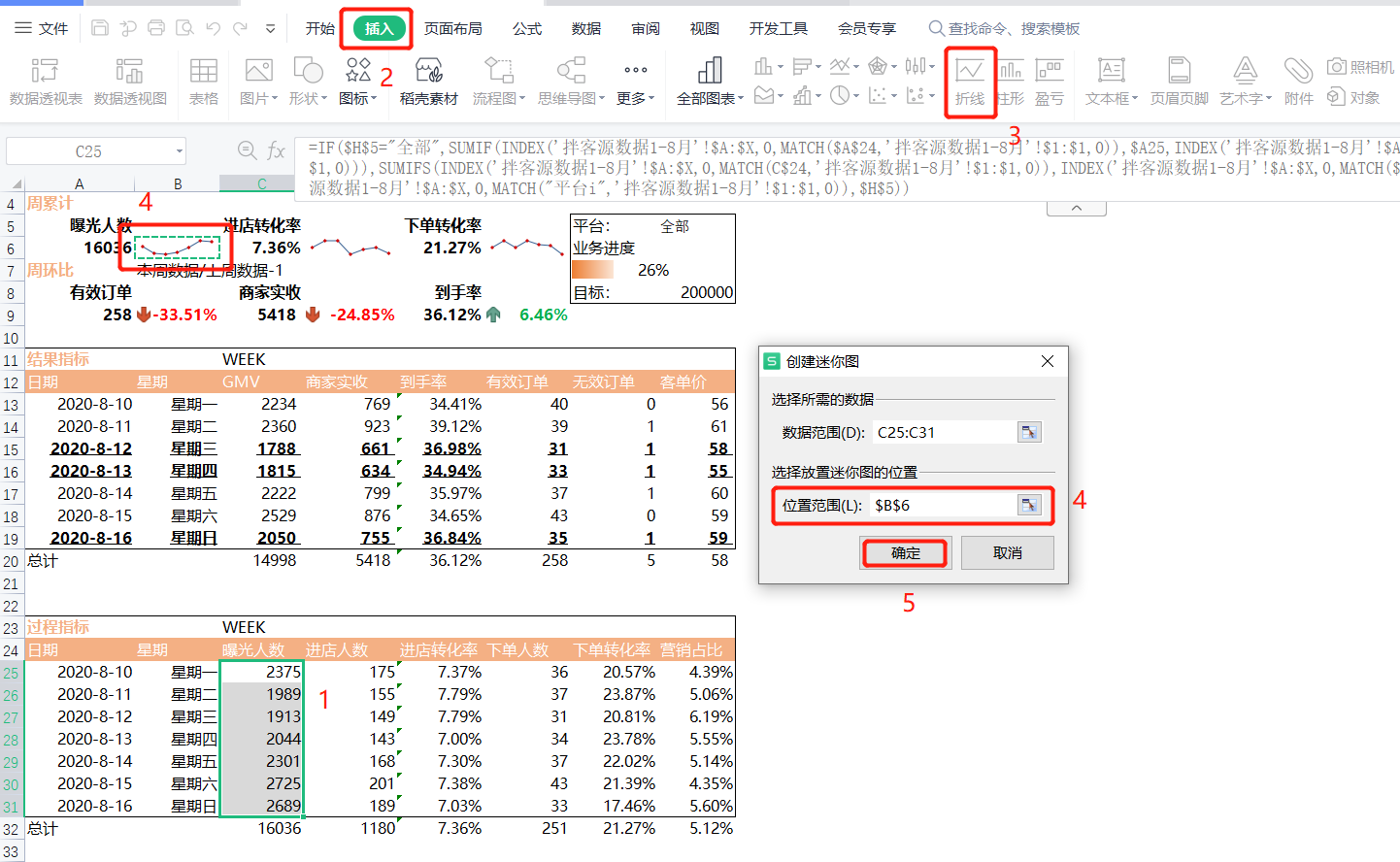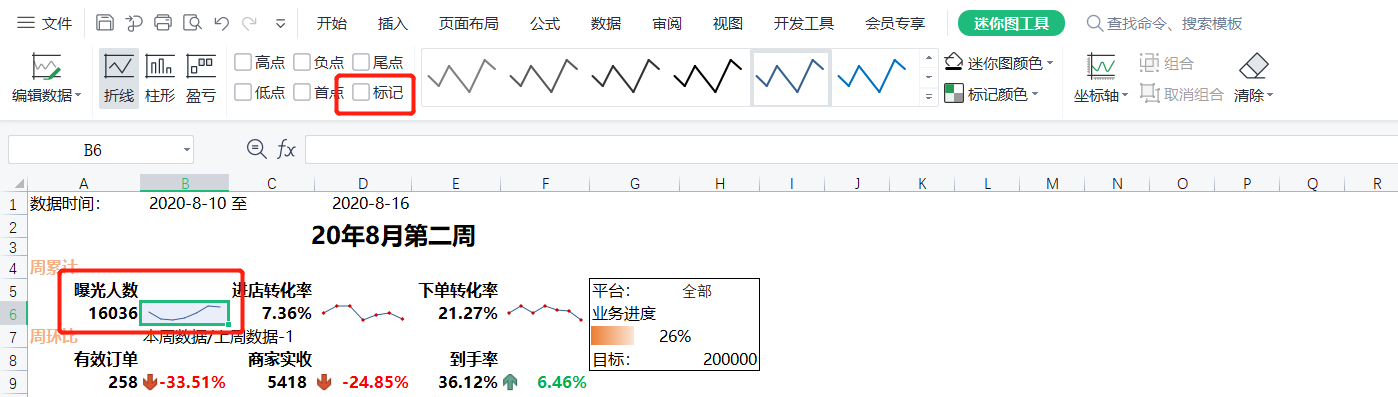## 6.同比/环比计算

周环比=本周数据/上周数据-1

小看板中周环比的本周有效订单、商家实收、到手率直接引用过程指标数据即可。
上周的数据可以通过日期偏移7天来求得，可以利用计算cpc总费用的总计来进行修改，逻辑如下：

if(平台=全部,sumifs(有效订单列,日期列,">="&开始日期-7,日期列,"<="&结束日期-7),sumifs(有效订单列,日期列,">="&开始日期-7,日期列-7,"<="&结束日期,平台列,平台))

• 上周的有效订单（F12单元格)完整函数实现
IF($H$5="全部",SUMIFS(INDEX('源数据表'!$A:$X,0,MATCH(F$12,'源数据表'!$1:$1,0)),INDEX('源数据表'!$A:$X,0,MATCH($A$12,'源数据表'!$1:$1,0)),">="&A13-7,INDEX('源数据表'!$A:$X,0,MATCH($A$12,'源数据表'!$1:$1,0)),"<="&$A$19-7),SUMIFS(INDEX('源数据表'!$A:$X,0,MATCH(F$12,'源数据表'!$1:$1,0)),INDEX('源数据表'!$A:$X,0,MATCH($A$12,'源数据表'!$1:$1,0)),">="&A13-7,INDEX('源数据表'!$A:$X,0,MATCH($A$12,'源数据表'!$1:$1,0)),"<="&$A$19-7,INDEX('源数据表'!$A:$X,0,MATCH("平台i",'源数据表'!$1:$1,0)),$H$5))

• 周环比有效订单完整函数实现(本周有效订单单元格A9)：
=A9/IF($H$5="全部",SUMIFS(INDEX('源数据表'!$A:$X,0,MATCH(F$12,'源数据表'!$1:$1,0)),INDEX('源数据表'!$A:$X,0,MATCH($A$12,'源数据表'!$1:$1,0)),">="&A13-7,INDEX('源数据表'!$A:$X,0,MATCH($A$12,'源数据表'!$1:$1,0)),"<="&$A$19-7),SUMIFS(INDEX('源数据表'!$A:$X,0,MATCH(F$12,'源数据表'!$1:$1,0)),INDEX('源数据表'!$A:$X,0,MATCH($A$12,'源数据表'!$1:$1,0)),">="&A13-7,INDEX('源数据表'!$A:$X,0,MATCH($A$12,'源数据表'!$1:$1,0)),"<="&$A$19-7,INDEX('源数据表'!$A:$X,0,MATCH("平台i",'源数据表'!$1:$1,0)),$H$5))-1

• 周环比商家实收的函数实现与周环比有效订单的函数实现逻辑一致，仅需把函数中的有效订单单元格A9改为商家实收单元格D12即可，完整函数实现如下（本周商家实收单元格C9）：
=C9/IF($H$5="全部",SUMIFS(INDEX('源数据表'!$A:$X,0,MATCH($D$12,'源数据表'!$1:$1,0)),INDEX('源数据表'!$A:$X,0,MATCH($A$12,'源数据表'!$1:$1,0)),">="&A13-7,INDEX('源数据表'!$A:$X,0,MATCH($A$12,'源数据表'!$1:$1,0)),"<="&$A$19-7),SUMIFS(INDEX('源数据表'!$A:$X,0,MATCH(D12,'源数据表'!$1:$1,0)),INDEX('源数据表'!$A:$X,0,MATCH($A$12,'源数据表'!$1:$1,0)),">="&A13-7,INDEX('源数据表'!$A:$X,0,MATCH($A$12,'源数据表'!$1:$1,0)),"<="&$A$19-7,INDEX('源数据表'!$A:$X,0,MATCH("平台i",'源数据表'!$1:$1,0)),$H$5))-1

• 上周的到手率需要通过上周的商家实收和上周GMV进行计算
到手率周环比=本周到手率/上周到手率-1
上周到手率=上周商家实收/上周GMV
>因此，
到手率环比=本周到手率/(上周商家实收/上周GMV)-1

• 上周的商家实收已在周环比商家实收中计算过，可直接复制函数使用
IF($H$5="全部",SUMIFS(INDEX('源数据表'!$A:$X,0,MATCH($D$12,'源数据表'!$1:$1,0)),INDEX('源数据表'!$A:$X,0,MATCH($A$12,'源数据表'!$1:$1,0)),">="&A13-7,INDEX('源数据表'!$A:$X,0,MATCH($A$12,'源数据表'!$1:$1,0)),"<="&$A$19-7),SUMIFS(INDEX('源数据表'!$A:$X,0,MATCH(D12,'源数据表'!$1:$1,0)),INDEX('源数据表'!$A:$X,0,MATCH($A$12,'源数据表'!$1:$1,0)),">="&A13-7,INDEX('源数据表'!$A:$X,0,MATCH($A$12,'源数据表'!$1:$1,0)),"<="&$A$19-7,INDEX('源数据表'!$A:$X,0,MATCH("平台i",'源数据表'!$1:$1,0)),$H$5))

• 上周的GMV与上周的商家实收的函数实现逻辑一致，仅需把函数中的商家实收单元格D12改为GMV单元格C12即可
IF($H$5="全部",SUMIFS(INDEX('源数据表'!$A:$X,0,MATCH($C$12,'源数据表'!$1:$1,0)),INDEX('源数据表'!$A:$X,0,MATCH($A$12,'源数据表'!$1:$1,0)),">="&A13-7,INDEX('源数据表'!$A:$X,0,MATCH($A$12,'源数据表'!$1:$1,0)),"<="&$A$19-7),SUMIFS(INDEX('源数据表'!$A:$X,0,MATCH($C$12,'源数据表'!$1:$1,0)),INDEX('源数据表'!$A:$X,0,MATCH($A$12,'源数据表'!$1:$1,0)),">="&A13-7,INDEX('源数据表'!$A:$X,0,MATCH($A$12,'源数据表'!$1:$1,0)),"<="&$A$19-7,INDEX('源数据表'!$A:$X,0,MATCH("平台i",'源数据表'!$1:$1,0)),$H$5))

• 周环比到手率完整函数实现（本周到手率单元格E9）：
=E9/(IF($H$5="全部",SUMIFS(INDEX('源数据表'!$A:$X,0,MATCH($D$12,'源数据表'!$1:$1,0)),INDEX('源数据表'!$A:$X,0,MATCH($A$12,'源数据表'!$1:$1,0)),">="&A13-7,INDEX('源数据表'!$A:$X,0,MATCH($A$12,'源数据表'!$1:$1,0)),"<="&$A$19-7),SUMIFS(INDEX('源数据表'!$A:$X,0,MATCH(D12,'源数据表'!$1:$1,0)),INDEX('源数据表'!$A:$X,0,MATCH($A$12,'源数据表'!$1:$1,0)),">="&A13-7,INDEX('源数据表'!$A:$X,0,MATCH($A$12,'源数据表'!$1:$1,0)),"<="&$A$19-7,INDEX('源数据表'!$A:$X,0,MATCH("平台i",'源数据表'!$1:$1,0)),$H$5))/IF($H$5="全部",SUMIFS(INDEX('源数据表'!$A:$X,0,MATCH($C$12,'源数据表'!$1:$1,0)),INDEX('源数据表'!$A:$X,0,MATCH($A$12,'源数据表'!$1:$1,0)),">="&A13-7,INDEX('源数据表'!$A:$X,0,MATCH($A$12,'源数据表'!$1:$1,0)),"<="&$A$19-7),SUMIFS(INDEX('源数据表'!$A:$X,0,MATCH($C$12,'源数据表'!$1:$1,0)),INDEX('源数据表'!$A:$X,0,MATCH($A$12,'源数据表'!$1:$1,0)),">="&A13-7,INDEX('源数据表'!$A:$X,0,MATCH($A$12,'源数据表'!$1:$1,0)),"<="&$A$19-7,INDEX('源数据表'!$A:$X,0,MATCH("平台i",'源数据表'!$1:$1,0)),$H$5)))-1


## 7.进度条

1)添加目标
当平台等于全部时，目标为20W；当平台等于美团时，目标为15W；当平台为饿了么时，目标为5W。函数实现如下

=IF($H$5="全部",200000,IF($H$5="美团",150000,50000))


2)计算业务进度

业务进度=截至目前整个月的GMV/目标
截至目前整个月的GMV=本月的第一天至本周的最后一天的GMV/目标

• 利用DATE、YEAR、MONTH函数求本月的第一天（本周第一天单元格A13）
DATE(YEAR($A$13),MONTH($A$13),1)

• 求本月第一天至本周最后一天GMV与求上周GMV逻辑一致，将日期判断修改为大于等于本月第一天，小于等于本周最后一天即可，完整函数实现如下：
IF($H$5="全部",SUMIFS(INDEX('源数据表'!$A:$X,0,MATCH($C$12,'源数据表'!$1:$1,0)),INDEX('源数据表'!$A:$X,0,MATCH($A$12,'源数据表'!$1:$1,0)),">="&DATE(YEAR($A$13),MONTH($A$13),1),INDEX('源数据表'!$A:$X,0,MATCH($A$12,'源数据表'!$1:$1,0)),"<="&$A$19),SUMIFS(INDEX('源数据表'!$A:$X,0,MATCH($C$12,'源数据表'!$1:$1,0)),INDEX('源数据表'!$A:$X,0,MATCH($A$12,'源数据表'!$1:$1,0)),">="&DATE(YEAR($A$13),MONTH($A$13),1),INDEX('源数据表'!$A:$X,0,MATCH($A$12,'源数据表'!$1:$1,0)),"<="&$A$19,INDEX('源数据表'!$A:$X,0,MATCH("平台i",'源数据表'!$1:$1,0)),$H$5))


3)添加进度条
菜单栏【开始】→功能区【条件格式】→【新建规则】→格式样式【数据条】→最小值/最大值【数字】→值【0】至【1】→选择填充类型、填充颜色→【确定】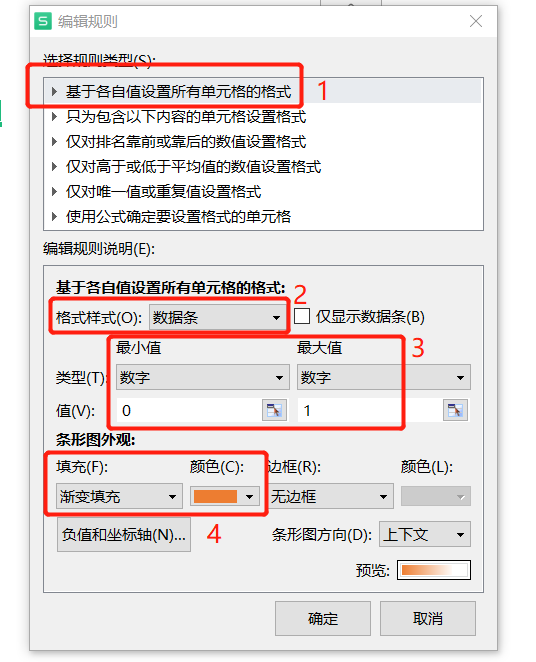## 8.图标指示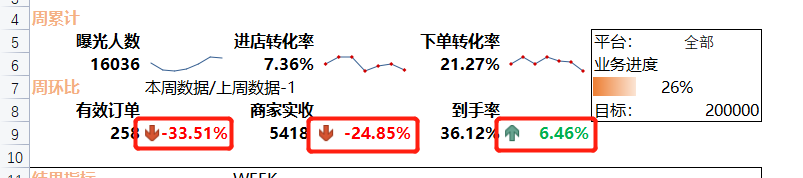为小看板中的周环比添加图标指示。正数显示为绿色，同时绿色箭头向下；负数或零时显示为红色，同时红色箭头向下。设置条件格式如下：
菜单栏【开始】→功能区【条件格式】→【新建规则】→选择规则类型【只包含一下内容的单元格设置格式】→【单元格值】【大于】【=0】→【格式】→【字体】→【颜色】

• 分别设置字体当数值大于0绿色、小于等于0黄色：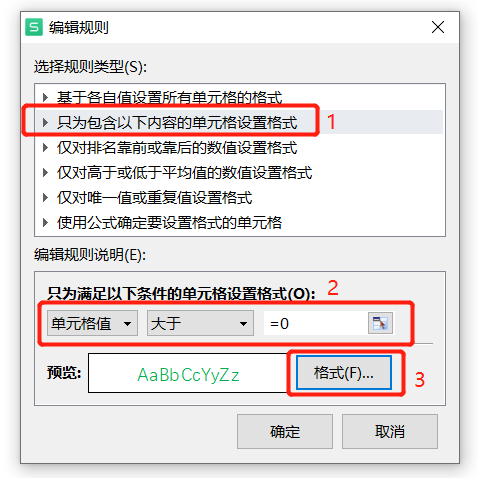• 设置图标
菜单栏【开始】→功能区【条件格式】→【新建规则】→格式样式【图标集】→选择对应图标→值【>=】【0】→类型【数字】→【确定】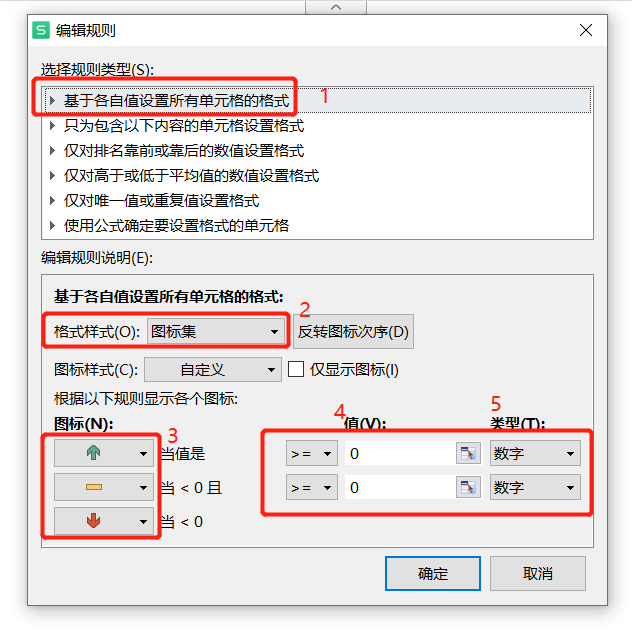所有条件设置如下，利用格式刷使该条件格式应用在其他环比数据上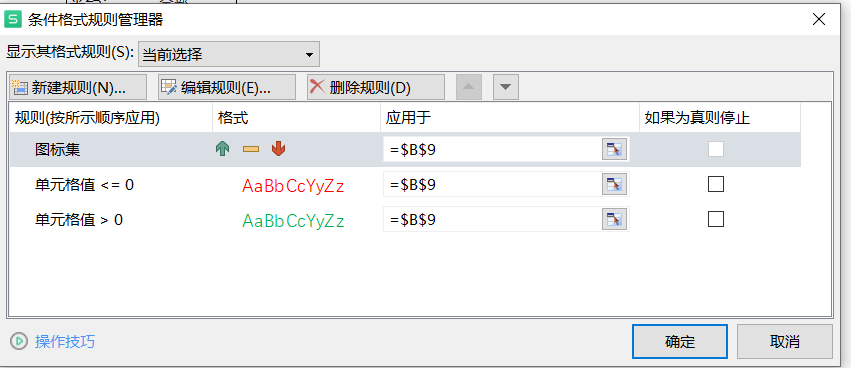## 9.突出显示

• 标记结果指标中GMV低于平均值的数据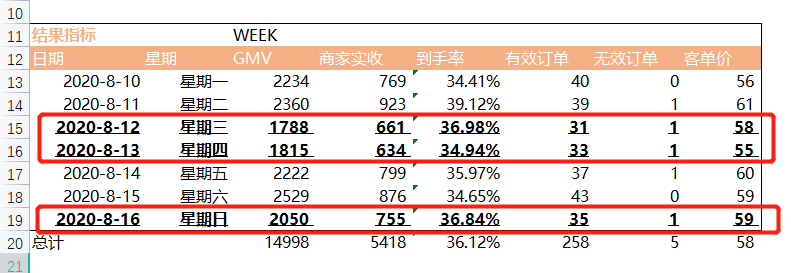选中条件判断区域→菜单栏【开始】→功能区【条件格式】→【新建规则】→选择规则类型【使用公式确定要设置的单元格】→设置公式，判断C13-C19整列数据→【格式】→【字体】→下划线【单下划线】→字形【粗体】→【确定】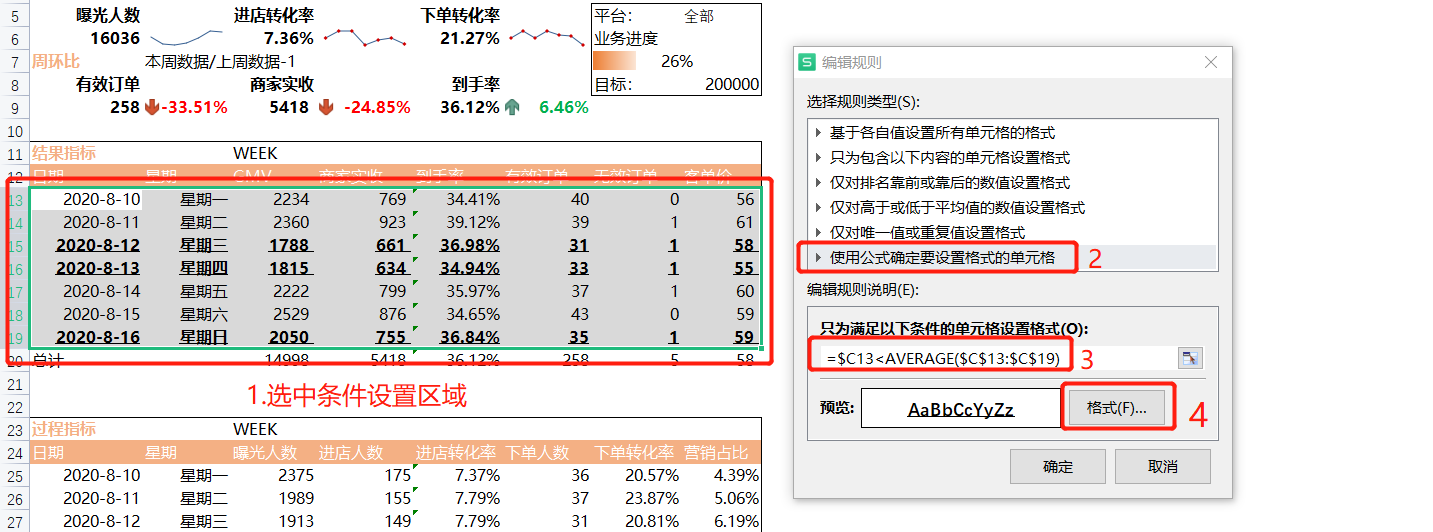## 10.细节美化

1)标题居中、字体加粗放大
2)关键字、关键数据加粗
3)表头添加背景颜色、修改字体颜色
4)添加外框线

展开全文经验分享
• 主要介绍了python实现自动化报表(Oracle/plsql/Excel/多线程)的相关知识，本文给大家介绍的非常详细，具有一定的参考借鉴价值,需要的朋友可以参考下
• 全文共2391字，预计学习时长14分钟 图源：morioh 工作中，无穷无尽的表格有时会令人抓狂。...用Python将Excel报表自动化 你还在天天做Excel报表吗？而且还是为不同的客户做着四五次同样的报表吗...

全文共2391字，预计学习时长14分钟图源：morioh

工作中，无穷无尽的表格有时会令人抓狂。Excel无处不在，即便有着像Python这样的强大工具任你使用，你也难以从中逃脱。

也许你的老板和同事仍然需要这种简单的方法来访问重要数据。但没关系！那并不意味着你不能通过使用Python来简化Excel工作。

全程无需使用Excel，逃离报表魔爪！

用Python将Excel报表自动化

你还在天天做Excel报表吗？而且还是为不同的客户做着四五次同样的报表吗？不如让Python代劳吧！

通过使用笔者在数据透视表教程中的数据（
https://towardsdatascience.com/a-step-by-step-guide-to-pandas-pivot-tables-e0641d0c6c70），概览一下我们将要自动化到底是什么。

数据格式将使你期望从公司数据库中获得的内容与客户销售数据相匹配，与你期望从公司数据库中获得的数据相匹配。它按区域细分这些数据，并且创建两个带有格式和图表的汇总表。无需使用Excel！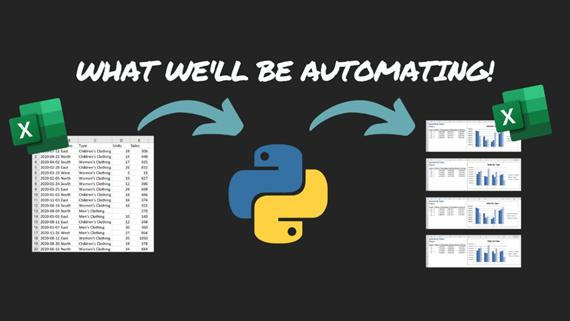最终的数据流—一个工作簿转化为四个格式化报告 | 图源：Nik Piepenbreier

加载库

使用Pandas和Openpyxl。笔者的“用Python自动化这三项（无聊！！！）Excel任务”（

#Section 1 - Loading our Libraries
import pandas as pd
from openpyxl.styles importFont
from openpyxl.chart importBarChart, Reference

你使用如下两个Librariy:

1. Pandas负责转化数据，并创建初始Excel文件

2. Openpyxl将工作薄格式化，并插入图表

加载数据

加载数据，并概览正在处理的内容。正如笔者所提到的，这些数据与读者从公司数据库系统获得的数据相似。

#Section 2 - Loading our Data
#        Date Region                 Type  Units Sales
#0 2020-07-11   East Children's Clothing   18.0    306
#1 2020-09-23  North Children's Clothing   14.0    448
#2 2020-04-02  South    Women's Clothing   17.0    425
#3 2020-02-28   East Children's Clothing   26.0    832
#4 2020-03-19   West    Women's Clothing    3.0     33

在这里，使用Pandas读取Excel文件，并读取Date/日期列的日期。

创建数据透视表

接着我们要创建最终报告中所需要的汇总表。这里将创建一个数据透视表以作为例子，其中仅使用东部地区进行代码的实验。

#Section 3 - Testing Pivot Tables
filtered= df[df['Region'] =='East']
quarterly_sales= pd.pivot_table(filtered, index =filtered['Date'].dt.quarter, columns ='Type', values ='Sales', aggfunc='sum')
print("Quarterly Sales Pivot Table:")
#Quarterly Sales Pivot Table:
#Type Children's Clothing  Men'sClothing  Women's Clothing
#Date
#1                   12274           13293             16729
#2                    5496           17817             22384
#3                   14463            9622             15065
#4                  13616           10953             16051

创建第一个Excel文件

有了数据透视表后，将其导入到一个Excel文件中，我们将用pandas来导入：

#Section 04 - Creating and Excel Workbook
file_path=#Path to where you want your file saved
quarterly_sales.to_excel(file_path, sheet_name ='Quarterly Sales', startrow=3)

这一步是在做什么：

· 创建一个文件路径变量，以确定要将文件存储在何处，

· 使用ExcelWriter保存文件

· 将两个透视表保存到单独的工作表中，从第3行开始（稍后从中保留以用于页眉）

使报表更漂亮

Pandas有助于将数据导入到Excel中。既然数据已经导入Excel，不妨将其美化一下，来添加一些可视化效果。

#Section 05 - Loading the Workbook
sheet1= wb['Quarterly Sales']
# Section 06 - Formatting the First Sheet
sheet1['A1'] ='Quarterly Sales'
sheet1['A2'] ='datagy.io'
sheet1['A4'] ='Quarter'
sheet1['A1'].style ='Title'
for i inrange(5, 9):
sheet1[f'B{i}'].style='Currency'
sheet1[f'C{i}'].style='Currency'
sheet1[f'D{i}'].style='Currency'
# Section 07 - Adding a Bar Chart
bar_chart=BarChart()
data=Reference(sheet1, min_col=2, max_col=4, min_row=4, max_row=8)
categories=Reference(sheet1, min_col=1, max_col=1, min_row=5, max_row=8)
bar_chart.set_categories(categories)
bar_chart.title ='Sales by Type'
bar_chart.style=3
wb.save(filename = file_path)

在Section 5中，将工作簿和工作表加载到Openpyxl可以处理的单独对象中。

而Section 6中操作更多：

· 在表一的A1和A2单元格中添加标题和副标题。

· 更改“quarters”列的标题，使其更能反映数据。

· 对标题和副标题应用样式。

· 将金融领域的单元格转换为货币。这需要对每单个单元格进行单独处理。因此使用了for循环。

在Section 7中，添加了条形图：

· 创建一个条形图对象，并识别存储数据和类别的字段。

· 随后将数据和类别应用于对象。

· 最后，添加描述性标题和样式。使用许多不同的样式都试试！

这就是工作簿现在的样子：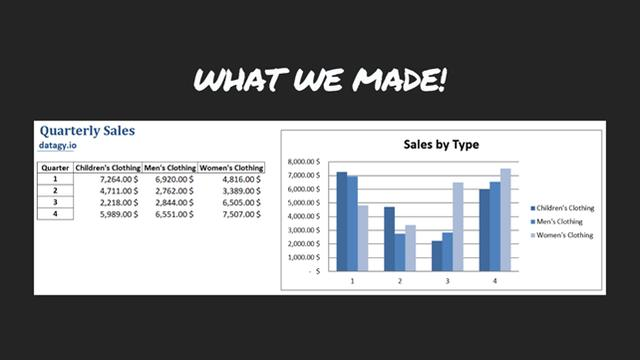所得工作簿之一 | 图源: Nik Piepenbreier

对多个工作簿执行工作流自动化

虽然已经很方便了，但是仅在一个区域执行这样的操作只能节约一点点的时间。我们可使用for循环，对所有的区域执行此操作。

#Section 08 - Getting Region Names
regions =list(df['Region'].unique())
# Section 09 - Looping Over All Regions
folder_path=#Insert the path to the folder you want tosave the reports in
for region in regions:
filtered =df[df['Region'] ==f'{region}']
quarterly_sales = pd.pivot_table(filtered, index =filtered['Date'].dt.quarter, columns ='Type', values ='Sales', aggfunc='sum')
quarterly_sales.to_excel(file_path,sheet_name ='QuarterlySales', startrow=3)
sheet1 = wb['Quarterly Sales']
sheet1['A1'] ='Quarterly Sales'
sheet1['A2'] ='datagy.io'
sheet1['A4'] ='Quarter'
sheet1['A1'].style ='Title'
for i inrange(5, 10):
sheet1[f'B{i}'].style='Currency'
sheet1[f'C{i}'].style='Currency'
sheet1[f'D{i}'].style='Currency'
bar_chart =BarChart()
data =Reference(sheet1, min_col=2, max_col=4, min_row=4, max_row=8)
categories =Reference(sheet1, min_col=1, max_col=1, min_row=5, max_row=8)
bar_chart.set_categories(categories)
bar_chart.title='Sales by Type'
bar_chart.style =3

在Section 8中，创建了一个列表，其中包含了想要覆盖的不同区域的所有唯一值。

在Section 9中，在for循环中重复先前的代码：

· 创建一个新变量，该变量用于保存文件所在文件夹的路径

· 接下来，使用f-strings将区域名插入到脚本中，使得脚本对每个区域都是动态的。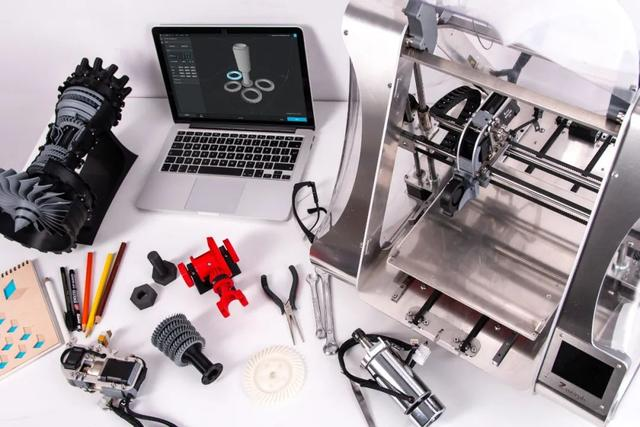图源：unsplash

Python的好处在于，它可使重复的任务具有可伸缩性。

想象一下，如果你每天都会收到这份文件，并且每天都要创建这些工作簿。这种方法能帮你节省多少时间！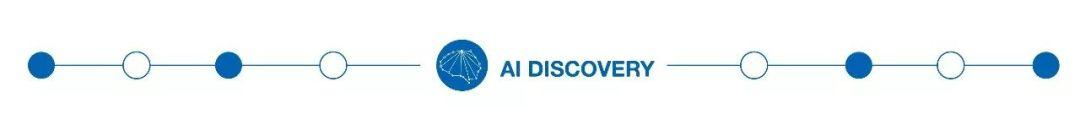我们一起分享AI学习与发展的干货
欢迎关注全平台AI垂类自媒体 “读芯术”（添加小编微信：dxsxbb，加入读者圈，一起讨论最新鲜的人工智能科技哦～）

展开全文python
• 我是小z今天给大家分享一篇俊红新书《对比Excel，轻松学习Python报表自动化》中关于报表自动化实战的内容，文末也会免费赠送几本新书。这本书是俊红新作，强烈安利！这篇文章将带你了解报表自动化的流程，并教你用...python
• 主要介绍了python实现报表自动化详解，涉及python读，写excel—xlwt常用功能，xlutils 常用功能，xlwt写Excel时公式的应用等相关内容，具有一定参考价值，需要的朋友可以了解下。
• 一、录制宏的基础操作：1 新建sheet；2 打开、保存和查看方式；3 关闭屏幕刷新；4 使用相对引用。 二、实行for 循环。 三、练习案例：练习1 - FOR 循环；练习2 - vlookup自动化
• 比如如何实现自动化，需要用到哪些函数 3、建立数据源表 4、建立数据转化表 也就是可以动态变化的表格 5、按照设计酌，逐个添加报告元素 6、调整格式 先看一下最终的形式 数据源表 数据转化表 正文...
• Excel数据分析与处理第十三章01Power Query02Power Pivot目录CONTENTS14.1 Power Query概述Power BI是一套强大的商业智能分析及数据可视工具能快速地将复杂的原始数据组织成直观有效的数据图表使用户能根据图表...
• ## Python-Excel报表自动化生成报表(一)

万次阅读 多人点赞 2019-06-12 16:48:41
使用python语言，实现对excel工作簿表格的自动化处理，自动生成各类统计报图表，让定期反复的报表处理工作变得简单快捷。excel
• 大家好，今天给大家分享如何轻松用 Python 制作自动化报表，喜欢记得收藏、点赞、关注。 ⚠️注意：文末提供技术交流群，完整版代码文末获取 本篇文章将带你了解报表自动化的流程，并教你用Python实现工作中的一个...python 时间序列
• 1.让Excel连接数据库，一键刷出明细数据，秒出报表。 2.把报表发布到web，方便团队共享； 3.能控制数据权限，用户只能看到自己的数据。 插件支持excel 2010/2013/2016/2019, 也支持wps。 适合各行业做报表的表哥...
• 问题描述：Excel数据更新繁琐虽然市面上各类报表工具，可视工具种类繁多，比如Tableau，Power BI，FineBI 等等，但是Excel 依旧是日常数据工作中的主力，我们依旧使用Excel 来制作各类数据报表，绘制各种图表，...
• 这是免费系列教程《7天学会商业智能(BI)-Power BI》的最后一篇内容：制作完的报表，如何发布？主题内容第1天：什么是报表？什么是报表？如何设计报表？第2天：认识Power BI什么...项目管理 github 软件测试
• 本文实例讲述了Python实现定制自动化业务流量报表周报功能。分享给大家供大家参考，具体如下： 一 点睛 本次实践通过定制网站5个频道的流量报表周报，通过XlsxWriter 模块将流量数据写入Excel文档，同时自动计算各...
• python实实现现报报表表自自动动化化详详解解 这篇文章主要介绍了python实现报表自动化详解涉及python读写excelxlwt常用功能xlutils 常用功能xlwt 写Excel时公式的应用等相关内容具有一定 考价值需要的朋友可以了解...
• 摘要:Delphi源码,报表打印,Delphi表格　Delphi编写的Excel表格自动化控制器，需要Office软件中的Exce编程控件支持，因此编译前请先准备好支持库文件，编译时会有提示的。
• 公众号后台回复“图书“，了解更多号主新书内容 作者：小F 来源：法纳斯特 之前小F分享了不少关于Python自动化操作Excel的文章，大家都挺喜欢的。所以今天就带大家来实战一波，使用Py...python
• 接上个资源，可视图表素材 Excel模板办公表格多彩数据自动生成 ，一共100套，更多精彩，职场人士人手必备一份，万能图表素材，输入数据就可以自动生成，提高办公效率，升职加薪必备神器！
• 合并报表excel自动模板 介绍 (Intro) One day we decided that we don’t want to waste any more time on tasks that can be automated and we decided to start with automation of our merging process. 有一天，...python git
• 实现上面的自动化报表，也很简单。只要弄清楚三个问题。 报表怎么取数 信息化建设成熟一点的公司呢，数据都会上传到业务系统中，想用的时候直接取数拿来用就行，还是很方便的。但遇到数据分散在不同的系统中，或者...运维
• 使用python语言，实现对excel工作簿表格的自动化处理，自动生成各类统计报图表，让定期反复的报表处理工作变得简单快捷。
• 利用Excel的VBA代码实现自动化“收集原始数据、汇总计算和报表”.doc文档
• 利用Excel的VBA代码实现自动化“收集原始数据、归纳计算和报表”.doc文档...